# JP4894595B2 - Image processing apparatus and method, and program - Google Patents

## Info

Publication number
JP4894595B2
JP4894595B2 JP2007105540A JP2007105540A JP4894595B2 JP 4894595 B2 JP4894595 B2 JP 4894595B2 JP 2007105540 A JP2007105540 A JP 2007105540A JP 2007105540 A JP2007105540 A JP 2007105540A JP 4894595 B2 JP4894595 B2 JP 4894595B2
Authority
JP
Japan
Prior art keywords
value
input
pixel
unit
contrast component
Prior art date
Legal status (The legal status is an assumption and is not a legal conclusion. Google has not performed a legal analysis and makes no representation as to the accuracy of the status listed.)
Expired - Fee Related
Application number
JP2007105540A
Other languages
Japanese (ja)
Other versions
JP2008263475A (en
Inventor

Original Assignee
ソニー株式会社
Priority date (The priority date is an assumption and is not a legal conclusion. Google has not performed a legal analysis and makes no representation as to the accuracy of the date listed.)
Filing date
Publication date
Application filed by ソニー株式会社 filed Critical ソニー株式会社
Priority to JP2007105540A priority Critical patent/JP4894595B2/en
Publication of JP2008263475A publication Critical patent/JP2008263475A/en
Application granted granted Critical
Publication of JP4894595B2 publication Critical patent/JP4894595B2/en
Expired - Fee Related legal-status Critical Current
Anticipated expiration legal-status Critical

• 238000004364 calculation methods Methods 0.000 claims description 210
• 238000000034 methods Methods 0.000 claims description 114
• 239000000284 extracts Substances 0.000 claims description 12
• 238000003672 processing method Methods 0.000 claims description 12
• 238000006243 chemical reactions Methods 0.000 description 135
• 230000000875 corresponding Effects 0.000 description 33
• 230000001629 suppression Effects 0.000 description 13
• 230000002708 enhancing Effects 0.000 description 8
• 230000002441 reversible Effects 0.000 description 4
• 230000000694 effects Effects 0.000 description 3
• 239000000203 mixtures Substances 0.000 description 3
• 230000002238 attenuated Effects 0.000 description 1
• 230000003247 decreasing Effects 0.000 description 1

## Images

•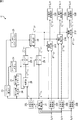••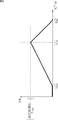•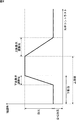•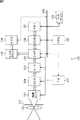•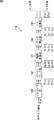•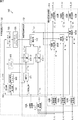•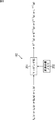•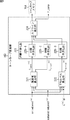•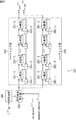•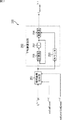••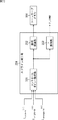•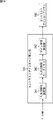•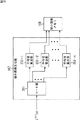•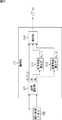•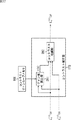•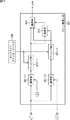•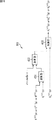•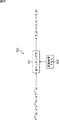•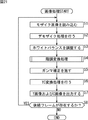•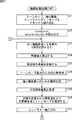•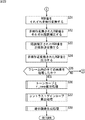•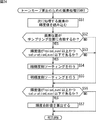•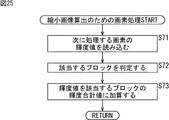•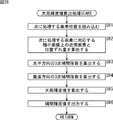•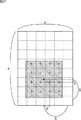•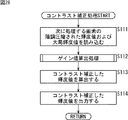•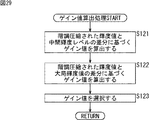•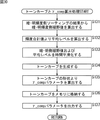•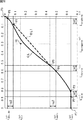•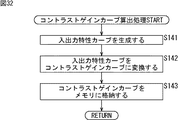•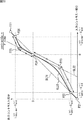•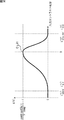•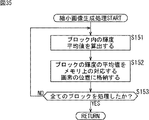•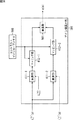•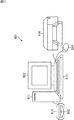•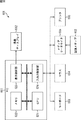•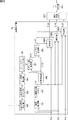•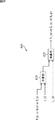•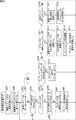•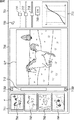•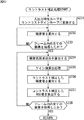•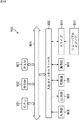## Classifications

• HELECTRICITY
• H04ELECTRIC COMMUNICATION TECHNIQUE
• H04NPICTORIAL COMMUNICATION, e.g. TELEVISION
• H04N9/00Details of colour television systems
• H04N9/64Circuits for processing colour signals
• H04N9/68Circuits for processing colour signals for controlling the amplitude of colour signals, e.g. automatic chroma control circuits
• HELECTRICITY
• H04ELECTRIC COMMUNICATION TECHNIQUE
• H04NPICTORIAL COMMUNICATION, e.g. TELEVISION
• H04N5/00Details of television systems
• H04N5/14Picture signal circuitry for video frequency region
• H04N5/20Circuitry for controlling amplitude response
• HELECTRICITY
• H04ELECTRIC COMMUNICATION TECHNIQUE
• H04NPICTORIAL COMMUNICATION, e.g. TELEVISION
• H04N5/00Details of television systems
• H04N5/14Picture signal circuitry for video frequency region
• H04N5/20Circuitry for controlling amplitude response
• H04N5/202Gamma control
• HELECTRICITY
• H04ELECTRIC COMMUNICATION TECHNIQUE
• H04NPICTORIAL COMMUNICATION, e.g. TELEVISION
• H04N9/00Details of colour television systems
• H04N9/64Circuits for processing colour signals
• H04N9/68Circuits for processing colour signals for controlling the amplitude of colour signals, e.g. automatic chroma control circuits
• H04N9/69Circuits for processing colour signals for controlling the amplitude of colour signals, e.g. automatic chroma control circuits for modifying the colour signals by gamma correction

## Description

The present invention relates to an image processing apparatus and method, and a program, and more particularly to an image processing apparatus and method suitable for use in correcting image contrast, and a program.

Image processing that reduces the gradation of an image and compresses the dynamic range is called gradation compression or gradation conversion. In recent years, various gradation compression or gradation conversion techniques have been proposed (for example, Patent Document 1).

Here, with reference to FIG. 1 to FIG. 3, the gradation conversion process performed in the invention described in Patent Document 1 will be described.

FIG. 1 is a block diagram illustrating an example of a functional configuration of a gradation conversion unit 11 that performs gradation conversion processing in the invention described in Patent Document 1. In FIG. The gradation conversion unit 11 includes a luminance calculation unit 21, nonlinear conversion units 22L to 22B, a tone curve calculation unit 23, a tone curve memory 24, a γ_comp parameter memory 25, a reduced image generation unit 26, a reduced image memory 27, an interpolation unit 28, The mapping units 29-1 and 29-2, the contrast correction unit 30, the gradation correction units 31R to 31B, and the non-linear inverse conversion units 32R to 32B are configured.

The tone conversion unit 11 performs tone compression processing for compressing the tone of the image using the tone curve and contrast correction processing for correcting the contrast lost by the tone compression processing. Of these, the tone compression processing is performed by the tone curve calculation unit 23, the tone curve memory 24, and the mapping units 29-1 and 29-2, and the contrast correction processing is performed by the γ_comp parameter memory 25, the reduced image generation unit 26, and the reduction. This is performed by the image memory 27, the interpolation unit 28, and the contrast correction unit 30.

Among them, in contrast correction processing, a low-frequency filter realized by a combination of image reduction processing by the reduced image generation unit 26 and image interpolation processing by the interpolation unit 28 is used to convert a component of an image subjected to gradation compression to a very low frequency component. Thus, the contrast correction is performed on the contrast component including not only the high-frequency component of the image but also the low and medium frequency components. Accordingly, it is possible to perform very natural contrast correction even if it looks visually without emphasizing only the details of the image.

FIG. 2 is a block diagram illustrating an example of a functional configuration of the contrast correction unit 30. The contrast correction unit 30 includes a gain value calculation unit 51 and a contrast enhancement unit 52.

The luminance value L c (nl) (p) of the image after gradation compression input to the contrast correction unit 30 has a contrast component compressed by a tone curve. The degree of compression depends on the inclination of the tone curve, and the degree of compression increases as the inclination becomes gentler. Therefore, in order to restore the contrast of the image, it is desirable to enhance the contrast component according to the reciprocal of the slope of the tone curve. However, in such a case, in the vicinity of the saturation level and the noise level of the luminance value, the contrast correction is too strong and the luminance saturation occurs, and black and white blurring may occur in the corrected image.

Therefore, in the contrast correction unit 30, the gain value calculation unit 51 uses the gain characteristic shown in FIG. 3 and the gain value g (p) based on the luminance value L c (nl) (p) after gradation compression. The contrast emphasizing unit 53 corrects the contrast using the gain value g (p) obtained by the gain value calculating unit 51. The horizontal axis direction of the graph of FIG. 3 represents the luminance value L c (nl) (p) after gradation compression, and the vertical axis direction represents the gain value g (p).

In the gain characteristic shown in FIG. 3, the luminance value L c (nl) (p) is a predetermined intermediate luminance level L mid (nl) that is approximately in the middle of the range that the luminance value L c (nl) (p) can take. The gain value g (p) becomes the maximum, and the gain value becomes closer to the minimum value L min (nl) or the maximum value L max (nl) of the range that the luminance value L c (nl) (p) can take. g (p) decays linearly, and in the range where the luminance value L c (nl) (p) is less than the minimum value L min (nl) and exceeds the maximum value L max (nl) , the gain value g (p) is 0. The gain value g (p) at the intermediate luminance level L mid (nl) is determined by a predetermined constant contrastGain and a γ_comp parameter determined by the shape of the tone curve, and becomes contrastGain / γ_comp−1. This gain characteristic can be expressed by the following equations (1) and (2).

The contrast enhancement unit 52 uses the gain value g (p) calculated by the gain value calculation unit 51 to calculate the contrast-corrected luminance value L u (nl) (p) by the following equation (3).

L u (nl) (p) = (g (p) +1) ・ (L c (nl) (p) −L cl (nl) (p)) + L cl (nl) (p)
＝ g (p) ・ (L c (nl) (p) −L cl (nl) (p)) + L c (nl) (p) (3)

L cl (nl) (p) represents the overall luminance value of the pixel at the pixel position p.

Therefore, the contrast of the luminance component near the intermediate luminance level L mid (nl) is enhanced as compared with the contrast of the luminance component near the minimum value L min (nl) or the maximum value L max (nl) . Strictly speaking, the contrast is emphasized as the luminance component near the intermediate luminance level L mid (nl) , and the contrast of the luminance component near the minimum value L min (nl) or the maximum value L max (nl) is almost enhanced. Not done. As a result, in the image after contrast correction, the luminance is saturated and the occurrence of white and black blurring is prevented.

The image contrast correction technique is also used for purposes other than correcting the contrast of an image obtained by tone-compressing an image having a wide dynamic range. For example, in the field of photo-retouching, when an image taken with a camera is not in focus, contrast correction is performed to emphasize the contrast component in the high frequency range, and the image is in focus. In general, the correction to be close is performed, and most of the photo-retouching software that provides a photo retouching function implements the correction function.

By the way, processing for enhancing the high-frequency contrast of this type of image is often realized by image processing called unsharp masking. Unsharp masking calculates the difference between the pixel value and the weighted average value of neighboring pixel values for each pixel of the image, and multiplies the difference value by an appropriate gain value to add it to the pixel value. It is processing. Here, the weighted average value of the neighboring pixel values corresponds to the low-frequency component in the pixel, and the high-frequency contrast component of the pixel is extracted by subtracting it from the pixel value. The gain value is a parameter for enhancing the extracted contrast component.

Further, some unsharp masking processes implemented in conventional photo retouching software have a function of appropriately adjusting the gain value so as to obtain a desired effect.

For example, conventionally, photo retouching software that can set a lower limit value of a contrast component to which contrast enhancement is applied as a threshold value is commercially available. In this software, when the high-frequency contrast component of the pixel is greater than or equal to the threshold value, the contrast component is emphasized with the specified gain value. When the contrast component is less than the threshold value, the gain value is set to 0, and the contrast component Is no longer emphasized. As a result, it is possible to suppress the enhancement of the high-frequency noise component together while enhancing the contrast component.

Conventionally, photo retouching software capable of setting the gain value characteristics in more detail is commercially available. FIG. 4 is a graph showing the characteristics of the gain value of the unsharp masking process of the photo retouching software. The horizontal axis in FIG. 4 indicates the local contrast value of the image, and the vertical axis indicates the contrast component enhancement rate, that is, the gain value. In this software, as shown in FIG. 4, in addition to the lower limit value, parameters of an upper limit value, a lower limit gradual adjustment range, an upper limit gradual adjustment range, a global effect, and an effect can be set. In this way, by making it possible to set the upper limit value in addition to the lower limit value of the contrast component to which the contrast correction is applied, contrast enhancement is performed more than necessary, luminance saturation occurs in areas where contrast is strong, and contrast is reduced. It is possible to avoid an image that is too strong and glaring and uncomfortable.

JP 2007-49450 A

However, in the invention described in Patent Document 1, there is a possibility that gradation reversal occurs in which the magnitude relationship between the luminance values is reversed by contrast correction processing. Here, the conditions under which gradation inversion occurs will be considered.

The following equation (4) is obtained by differentiating both sides of the above equation (3) by L c (nl) .

Since attention is now paid to gradation inversion in the local region, the general luminance value L cl (nl) is considered as a constant in the equation (4). Further, in the expression (4), the display of (p) representing the pixel position of each variable is omitted.

In order to prevent local gradation inversion, an expression is used within a range of effective luminance values from the minimum value L min (nl) to the maximum value L max (nl) (hereinafter referred to as an effective luminance range). It is necessary to set the gain value g so that the value on the right side of (4) does not become 0 or less.

In the invention described in Patent Document 1, the characteristics of the gain value g are expressed by the above-described FIG. 3 and the expressions (1) and (2). Only a range where L c (nl) is brighter than the intermediate luminance level L mid (nl) will be considered. Then, the gain value g in Expression (4) is expressed by the following Expression (5).

Here, g mid represents the peak value of the gain value g at the intermediate luminance level L mid (nl ), and is a constant represented by the following equation (6).

By substituting equation (5) into equation (4), the following equation (7) is obtained.

For example, when g mid = 1 and L cl (nl) = L mid (nl) , when L c (nl) = L max (nl) , the right side of equation (7) is 0. .

The value of g mid is almost determined by the shape of the histogram of the luminance value of the input image before gradation compression, and since the value of normal contrastGain is normally set to 1, in the case of a normal natural image, the value of g mid is empirical. It is known that it falls within the range of 0 to 1. Further, since the overall luminance value L cl (nl) is a luminance value of a very low frequency component of the image before contrast correction, it is rare that it becomes a value larger than L mid (nl) . Therefore, in a normal natural image, the monotonic increase of L u (nl) in the local region is almost guaranteed within the effective luminance range, and there is almost no possibility of gradation inversion.

However, if the contrastGain value is set to a value larger than 1 in order to enhance the contrast of the original input image for reasons such as improving the appearance of the image, g mid exceeds 1 even in a normal natural image. In some cases, the right side of Expression (7) becomes 0 or less in the range of L c (nl) <L max (nl) . This indicates that gradation inversion may occur in an image including a pixel having a large contrast component (L c (nl) −L cl (nl) ).

Also, in the gain characteristic of FIG. 4 described above, the gain attenuation characteristic on the side larger than the upper limit value is linear, similarly to the gain characteristic of FIG. 3, and for the same reason, gradation inversion can occur locally. There is sex.

Furthermore, in the invention described in Patent Document 1, when the value of contrastGain is set to a value larger than 1 and the gain value g (p) increases, the contrast component (L c (nl ) (p) −L cl (nl) (p)) is overemphasized and the luminance value L u (nl) (p) may exceed the black level luminance value or below the noise level luminance value. is there. That is, in the image after contrast correction, the luminance is saturated, and there is a possibility that white blur or black blur occurs.

For example, in the case of an image in which a high luminance region is spot-like in a low luminance region, the overall luminance value L cl (nl) (p) is small, so the contrast component is very large. In this case, when the contrastGain value is set to 1, as described above, the gain value g (p) does not exceed 1, and luminance saturation hardly occurs, but the contrastGain value is set to 1. When the value is set larger, the gain value g (p) exceeds 1, and as a result, the luminance is saturated in the image after contrast correction, and there is a possibility that white blur or black blur occurs.

In the photo retouching software having the gain characteristic shown in FIG. 4, the contrast correction is suppressed by the local contrast value and the saturation of the brightness is suppressed. For example, the contrast correction is performed on a high brightness region where the contrast component is not so strong. There is a possibility that the luminance is saturated when it is applied.

That is, in the invention described in Patent Document 1 and commercially available photo retouching software, there is a possibility that gradation inversion or luminance saturation may occur due to contrast correction depending on the image to be processed and the degree of enhancement of contrast. .

The present invention has been made in view of such a situation, and makes it possible to appropriately perform contrast correction according to each image.

An image processing apparatus according to a first aspect of the present invention is an image processing apparatus that extracts a contrast component obtained by removing low frequency components from an input image for each pixel and corrects the contrast component of each pixel based on a gain value. An input / output characteristic curve having an input contrast component, which is the contrast component before correction, as an input and an output contrast component, which is the contrast component after correction, as an output, wherein the output contrast with respect to the input contrast component Based on input / output characteristic generating means for generating an input / output characteristic curve whose component monotonically increases, and an inclination of a line segment connecting the origin and each point of the input / output characteristic curve, the input contrast component is used as the output contrast component. A gain for generating a gain characteristic curve representing the characteristic of the gain value for correction with respect to the input contrast component And sex generating means, for each pixel of the input image, and a gain value calculating means for calculating the gain value by applying the input contrast component of the pixel in the gain characteristic curve.

The input / output characteristic generating means has a maximum slope of greater than 1 when the value of the input contrast component is near 0, and the absolute value of the input contrast component is within a predetermined correction range. As the value increases, the inclination becomes gentler, and the absolute value of the output contrast component with respect to the input contrast component is equal to or greater than the absolute value of the input contrast component, and the inclination is within a range where the input contrast value exceeds the correction range. The input / output characteristic curve that is 1 or close to 1 can be generated.

An acquisition means for acquiring a maximum inclination that is an inclination of the input / output characteristic curve when the value of the input contrast component specified by the user is near 0 and the correction range is further provided; The input / output characteristic curve can be generated on the basis of the maximum slope and the correction range specified by.

The said gain value calculating means, for each pixel of the input image, said difference value between the intermediate luminance value is an intermediate value between the luminance value of the input image and the luminance value of the pixel can take the pixel The gain value can be calculated as an input contrast component .

The gain value calculation means can calculate the gain value for each pixel of the input image using the contrast component of the pixel as the input contrast component of the pixel .

The said gain value calculating means, for each pixel of the input image, said difference value between the intermediate luminance value is an intermediate value between the luminance value of the input image and the luminance value of the pixel can take the pixel is calculated first gain value as an input contrast component, wherein to calculate the second gain value a contrast component as the input contrast component of the pixel, the first gain value and the second gain value of the pixel By selecting the smaller one, the final third gain value can be calculated.
For each pixel of the input image, the gain value calculation means calculates a difference value between the luminance value of the pixel and an intermediate luminance value that is an intermediate value of a range that the luminance value of the input image can take. By calculating a first gain value as an input contrast component, calculating a second gain value using the contrast component of the pixel as the input contrast component, and multiplying the first gain value and the second gain value The final third gain value can be calculated.

An image processing method or program according to the first aspect of the present invention extracts, for each pixel, a contrast component obtained by removing a low-frequency component from an input image, and corrects the contrast component of each pixel based on a gain value. An image processing method of a processing device or a program that causes a computer to execute a process of extracting a contrast component obtained by removing low frequency components from an input image for each pixel and correcting the contrast component of each pixel based on a gain value. An input / output characteristic curve having an input contrast component, which is the contrast component before correction, as an input and an output contrast component, which is the contrast component after correction, as an output, wherein the output contrast with respect to the input contrast component generating output characteristic curve component increases monotonically, and the origin of the input-output characteristic curve Based on the slope of a line connecting the points, to generate a gain characteristic curve representing the characteristic for the input contrast component of the gain value for correcting the input contrast component to the output contrast component, the pixel of the input image Each step of calculating the gain value by applying the input contrast component of the pixel to the gain characteristic curve .

The image processing apparatus according to the second aspect of the present invention extracts, for each pixel, a contrast component obtained by removing a low frequency component from an input image, and the contrast of each pixel based on a gain value indicating a degree of emphasizing the contrast component. an image processing apparatus for correcting a component for each pixel of the input image, the first gain by applying the luminance value of the pixel in the first gain characteristic curve representing the characteristic of the gain value for the luminance value a first gain value calculating means for calculating a value for each pixel of the input image, by applying the contrast component of the pixel in the second gain characteristic curve representing the characteristic of the gain value for the contrast component a second gain value calculating means for calculating a second gain value, for each pixel of the input image, the first gain value and the second Comprising the smaller of the gain value, or a value obtained by multiplying the first gain value and the second gain value, and a third gain value calculating means for calculating as the final third gain value .

The image processing method or the program according to the second aspect of the present invention extracts, for each pixel, a contrast component obtained by removing a low frequency component from an input image, and sets each contrast value based on a gain value indicating the degree of enhancement of the contrast component. Based on the image processing method of the image processing apparatus for correcting the contrast component of the pixel, or the gain value indicating the degree of enhancement of the contrast component by extracting the contrast component obtained by removing the low frequency component from the input image for each pixel. a program for executing the process for correcting the contrast component of each pixel in a computer for each pixel of the input image, the luminance value of the pixel in the first gain characteristic curve representing the characteristic of the gain value for the luminance value the first gain value is calculated by applying, for each pixel of the input image, the contra The second gain value is calculated by applying the contrast component of the pixel in the second gain characteristic curve representing the characteristic of the gain value for the preparative components, for each pixel of the input image, the first gain A step of calculating a final third gain value by a smaller one of the value and the second gain value or a value obtained by multiplying the first gain value and the second gain value .

In the first aspect of the present invention, there is provided an input / output characteristic curve having an input contrast component, which is the contrast component before correction, as an input, and an output contrast component, which is the contrast component after correction, as an output. An input / output characteristic curve in which the output contrast component monotonously increases with respect to the contrast component is generated, and the input contrast component is converted to the output contrast based on a slope of a line segment connecting the origin and each point of the input / output characteristic curve. A gain characteristic curve representing a characteristic of the gain value for correcting the component with respect to the input contrast component is generated, and for each pixel of the input image, the input contrast component of the pixel is applied to the gain characteristic curve. The gain value is calculated.

In the second aspect of the present invention, for each pixel of the input image, the first gain value by applying the luminance value of the pixel in the first gain characteristic curve representing the characteristic of the gain value for the luminance value There is calculated for each pixel of the input image, the second gain value by applying the contrast component of the pixel in the second gain characteristic curve representing the characteristic of the gain value for the contrast component is calculated, A final third gain value is calculated for each pixel of the input image based on the first gain value and the second gain value.

As described above, according to the first aspect of the present invention, the gain value used for contrast correction can be controlled for each pixel. In addition, according to the first aspect of the present invention, it is possible to appropriately perform contrast correction according to each image so that gradation inversion does not occur.

According to the second aspect of the present invention, the gain value used for contrast correction can be controlled for each pixel. Further, according to the second aspect of the present invention, it is possible to appropriately perform contrast correction according to each image so that saturation of luminance does not occur.

Embodiments of the present invention will be described below. Correspondences between the constituent elements of the present invention and the embodiments described in the specification or the drawings are exemplified as follows. This description is intended to confirm that the embodiments supporting the present invention are described in the specification or the drawings. Therefore, even if there is an embodiment which is described in the specification or the drawings but is not described here as an embodiment corresponding to the constituent elements of the present invention, that is not the case. It does not mean that the form does not correspond to the constituent requirements. Conversely, even if an embodiment is described here as corresponding to a configuration requirement, that means that the embodiment does not correspond to a configuration requirement other than the configuration requirement. It's not something to do.

The image processing apparatus according to the first aspect of the present invention (for example, the gradation conversion unit 143 in FIG. 7 or the CPU 621 in FIG. 38) firstly applies a contrast component obtained by removing low frequency components from the input image for each pixel. An image processing apparatus that corrects the contrast component of each pixel based on a gain value, and receives an input contrast component that is the contrast component before correction, and outputs an output contrast that is the contrast component after correction An input / output characteristic curve having a component as an output, which generates an input / output characteristic curve in which the output contrast component monotonously increases with respect to the input contrast component (for example, the input / output characteristic curve CL21 in FIG. 33). and generating means (e.g., input-output characteristic setting unit 341 in FIG. 14, or input-output characteristic setting unit 643 in FIG. 39), the input-output characteristic curve Based on the slope of a line connecting the origin and each point, a gain characteristic curve of the input contrast component representing the characteristic for the input contrast component of the gain value for correcting the output contrast component (e.g., in FIG. 34 For each pixel of the input image, the gain characteristic generating means (for example, the gain characteristic calculating unit 343 in FIG. 14 or 39) that generates the contrast gain curve CL31) is used as the gain characteristic curve. Gain value calculation means (for example, gain value calculation unit 391 in FIG. 17 or 39) that calculates the gain value by applying is provided.

Secondly, the image processing apparatus according to the first aspect of the present invention acquires the maximum inclination and the correction range, which are specified by the user and are the slope of the input / output characteristic curve when the value of the input contrast component is near zero. Acquisition means (for example, the UI processing unit 633 in FIG. 39), and the input / output characteristic generation means generates the input / output characteristic curve based on the maximum slope and the correction range specified by a user. be able to.

An image processing method or program according to the first aspect of the present invention extracts, for each pixel, a contrast component obtained by removing a low-frequency component from an input image, and corrects the contrast component of each pixel based on a gain value. An image processing method, or a program that causes a computer to execute a process of extracting a contrast component obtained by removing a low frequency component from an input image for each pixel and correcting the contrast component of each pixel based on a gain value, An input / output characteristic curve having an input contrast component that is the contrast component before correction as an input and an output contrast component that is the contrast component after correction as an output, wherein the output contrast component is relative to the input contrast component Monotonically increasing input / output characteristic curve (for example, input / output characteristic curve CL2 in FIG. 33) ) Generate (e.g., step S141 in FIG. 32, or step S212 in FIG. 41), based on the inclination of a line connecting the respective points and the origin of the input-output characteristic curve, the output the input contrast component A gain characteristic curve (for example, contrast gain curve CL31 in FIG. 34) representing a characteristic of the gain value for correcting to the contrast component with respect to the input contrast component is generated (for example, step S142 in FIG. 32 or FIG. 43). In step S231), for each pixel of the input image, the gain value is calculated by applying the input contrast component of the pixel to the gain characteristic curve (for example, step S112 of FIG. 28 or step of FIG. 43). S235) including a step.

The image processing apparatus according to the second aspect of the present invention (for example, the gradation conversion unit 143 in FIG. 7 or the CPU 621 in FIG. 38) extracts, for each pixel, a contrast component obtained by removing low-frequency components from the input image. An image processing apparatus that corrects the contrast component of each pixel based on a gain value indicating a degree of emphasizing the contrast component, wherein the first value representing the characteristic of the gain value with respect to a luminance value for each pixel of the input image First gain value calculation means (for example, FIG. 18 or FIG. 36) that calculates the first gain value by applying the luminance value of the pixel to the gain characteristic curve (for example, the curve representing the gain characteristic of FIG. 3 ) . and the mapping unit 412-1), for each pixel of the input image, the second gain characteristic curve representing the characteristic of the gain value for the contrast component (e.g., FIG. Second gain value calculating means for calculating a second gain value by applying the contrast component of the pixel in the contrast gain curve CL31) of 4 (for example, the mapping unit 412-2 of FIG. 18 or 36) , A smaller one of the first gain value and the second gain value, or a value obtained by multiplying the first gain value and the second gain value by a final third gain value. Third gain value calculation means for calculating (for example, the selection unit 414 in FIG. 18 or the multiplication unit 501 in FIG. 36) is provided.

The image processing method or program according to the second aspect of the present invention extracts, for each pixel, a contrast component obtained by removing a low frequency component from an input image, and based on a gain value indicating the degree of enhancement of the contrast component. The image processing method of the image processing apparatus for correcting the contrast component, or each pixel based on the gain value indicating the degree of enhancement of the contrast component by extracting the contrast component obtained by removing the low frequency component from the input image for each pixel A first gain characteristic curve (for example, the gain characteristic of FIG. 3) that represents a characteristic of the gain value with respect to a luminance value for each pixel of the input image. calculating a first gain value by applying the luminance value of the pixel in the curve) representing the (e.g., 29 step S121 in), for each pixel of the input image, the second gain characteristic curve representing the characteristic of the gain value for the contrast component (e.g., the contrast component of the pixel in the contrast gain curve CL31) in FIG. 34 The second gain value is calculated by applying (for example, step S122 in FIG. 29), and for each pixel of the input image, the smaller of the first gain value and the second gain value, or A step of calculating a final third gain value by a value obtained by multiplying the first gain value and the second gain value (for example, step S123 in FIG. 29) is included.

Hereinafter, embodiments of the present invention will be described with reference to the drawings.

FIG. 5 is a block diagram showing an embodiment of a digital video camera to which the present invention is applied. The digital video camera 101 includes a lens 111, an aperture 112, an image sensor (CDS) 113, a correlated double sampling circuit (CDS) 114, an A / D converter 115, a DSP (Digital Signal Processor) block 116, and a timing generator (TG) 117. , An LCD (Liquid Crystal Display) driver 118, an LCD 119, a CODEC (Compression / Decompression) 120, a memory 121, a CPU 122, and an input device 123. The DSP block 116 is a block configured by a signal processing processor (for example, DSP) and a memory such as a RAM (Random Access Memory) that holds image data, and the processor executes a predetermined program. Thus, image processing to be described later is performed. Hereinafter, the DSP block 116 is simply referred to as the DSP 116.

Incident light from a subject that has passed through an optical system including the lens 111 and the diaphragm 112 first reaches each light receiving element on the imaging surface of the image sensor 113 and is converted into an electrical signal by photoelectric conversion by the light receiving element. The electrical signal output from the image sensor 113 is noise-removed by the correlated double sampling circuit 114, digitized by the A / D converter 115, and then the digitized image data is temporarily stored in the memory in the DSP 116. Stored. The timing generator 117 controls a signal processing system including the correlated double sampling circuit 114, the A / D converter 115, and the DSP 116 so that image data is captured at a constant frame rate. That is, the DSP 116 is supplied with a stream of image data at a constant frame rate.

Note that the image sensor 113 has a wider dynamic range than an image sensor such as a general CCD (Charge Coupled Device), and captures an image from a dark part to a bright part of a subject without being saturated or generating noise. be able to. Therefore, the A / D converter 115 has a greater number of gradations (for example, the number of gradations that can be expressed by data of about 10 to 12 bits) than the number of gradations of an ordinary digital video camera. , The number of gradations that can be expressed by data of about 14 to 16 bits).

The DSP 116 performs image processing, which will be described later, on the image data so that the dynamic range of the image data becomes, for example, a dynamic range that can be displayed by the LCD 119, and then converts the image data subjected to the image processing to the LCD as necessary. Supplied to driver 118 or CODEC 120.

The LCD driver 118 converts the image data supplied from the DSP 116 into an analog image signal. The LCD driver 118 supplies an analog image signal to the LCD 119 which is a finder of the digital video camera 101, and displays an image based on the image signal.

The CODEC 120 encodes image data supplied from the DSP 116 by a predetermined method, and records the encoded image data in a memory 121 made of a semiconductor, a magnetic recording medium, a magneto-optical recording medium, an optical recording medium, or the like.

The CPU 122 controls the overall processing of the digital video camera 101 based on, for example, a command input by the user by operating the input device 123 configured by operation buttons such as a shutter button.

FIG. 6 is a block diagram illustrating an example of a functional configuration realized when a processor (arithmetic unit) in the DSP 116 executes a predetermined program. Functions including the demosaic unit 141, the white balance unit 142, the gradation conversion unit 143, the gamma correction unit 144, and the YC conversion unit 145 are realized by the processor inside the DSP 116 executing a predetermined program.

The demosaic unit 141 acquires a mosaic image that is image data A / D converted by the A / D converter 115. A mosaic image is an image in which data corresponding to any color component of R, G, and B is stored in one pixel, and each pixel is arranged according to a color array called a Bayer array, for example. The demosaic unit 141 performs demosaic processing on a mosaic image so that one pixel has all the R, G, and B components. Thereby, three image data of R image, G image, and B image respectively corresponding to three color components of R, G, and B are generated. The demosaic unit 141 supplies the generated three image data of the R image, the G image, and the B image to the white balance unit 142.

Hereinafter, the three image data of the R image, the G image, and the B image are collectively referred to as an RGB image. Hereinafter, the pixel value at the pixel position p of the mosaic image is denoted by M (p). Further, hereinafter, the pixel value at the pixel position p of the demosaiced image data is assumed to be [R (p), G (p), B (p)]. R (p) is a pixel value of the R component, G (p) is a pixel value of the G component, and B (p) is a pixel value of the B component.

The white balance unit 142 adjusts the white balance of the RGB image so that the color balance of the achromatic portion of the subject actually becomes an achromatic color by applying an appropriate coefficient to each pixel value of the RGB image. The white balance unit 142 supplies the RGB image with the adjusted white balance to the gradation conversion unit 143. Hereinafter, the pixel value at the pixel position p of the image data whose white balance has been adjusted is referred to as [R w (p), G w (p), B w (p)]. R w (p) is the R component pixel value, G w (p) is the G component pixel value, and B w (p) is the B component pixel value.

As will be described later with reference to FIG. 22 and FIG. 23, the gradation conversion unit 143 performs gradation conversion processing on the RGB image whose white balance has been adjusted. The gradation conversion unit 143 supplies the RGB image subjected to the gradation conversion to the gamma correction unit 144. Hereinafter, the pixel value at the pixel position p of the image data subjected to the gradation conversion process is referred to as [R u (p), G u (p), B u (p)]. Note that R u (p) is the R component pixel value, G u (p) is the G component pixel value, and B u (p) is the B component pixel value.

The gamma correction unit 144 performs gamma correction on the RGB image that has undergone gradation conversion. The gamma correction unit 144 supplies the RGB image subjected to gamma correction to the YC conversion unit 145. Hereinafter, the pixel value at the pixel position p of the image data subjected to the gamma correction is referred to as [R u γ (p), G u γ (p), B u γ (p)]. Note that R u γ (p) is a pixel value of the R component, G u γ (p) is a pixel value of the G component, and B u γ (p) is a pixel value of the B component.

The YC conversion unit 145 performs YC matrix processing and band limitation on the chroma component on the RGB image subjected to gamma correction, thereby performing a Y image composed of luminance components (Y components) and a color difference component ( C image composed of Cb or Cr component is generated. The YC conversion unit 145 supplies the generated Y image and C image to the LCD driver 118 or the CODEC 120 as necessary. Hereinafter, the pixel value at the pixel position p of the image data output from the YC conversion unit 145 is referred to as [Y (p), C (p)]. Y (p) is the value of the luminance component in the Y image, and C (p) is the value of the color difference component in the C image.

FIG. 7 is a block diagram illustrating an example of a functional configuration of the gradation conversion unit 143. The gradation conversion unit 143 is configured to include a global information calculation unit 151, a luminance value pixel processing unit 152, and an RGB value pixel processing unit 153.

The global information calculation unit 151 generates a reduced image obtained by reducing the image composed of the luminance values of the RGB image, a tone curve for appropriately compressing the gradation of the luminance value of the RGB image, and the luminance value of the RGB image A contrast gain curve for appropriately correcting the contrast is generated (calculated), and the generated reduced image, tone curve, and contrast gain curve are supplied to the luminance value pixel processing unit 152.

The luminance value pixel processing unit 152 uses the reduced image, tone curve, and contrast gain curve generated by the global information calculation unit 151 to perform tone conversion and contrast correction on the luminance value of each pixel of the RGB image. The luminance value obtained as a result is supplied to the RGB value pixel processing unit 153.

The RGB value pixel processing unit 153 uses the luminance value calculated by the luminance value pixel processing unit 152 to correct the RGB value of each pixel of the RGB image.

The global information calculation unit 151 includes a luminance calculation unit 161, a non-linear conversion unit 162L, a tone curve calculation unit 163, a tone curve memory 164, a contrast gain curve calculation unit 165, a contrast gain curve memory 166, a reduced image generation unit 167, and a reduction An image memory 168 is included.

As will be described later with reference to FIG. 22, the luminance calculation unit 161 determines the pixel position from the pixel values R w (p), G w (p), and B w (p) of the RGB image whose white balance has been adjusted. The value of the luminance component corresponding to (luminance value L (p)) is calculated. The luminance calculation unit 161 outputs the calculated luminance value L (p) to the non-linear conversion unit 162L.

The non-linear conversion unit 162L performs non-linear conversion on the luminance value L (p) as described later with reference to FIG. The nonlinear conversion unit 162L converts the luminance value L (nl) (p) calculated by nonlinear conversion into a tone curve calculation unit 163, a reduced image generation unit 167, an interpolation unit 171, a mapping unit 172-1, and a gradation. The data is output to the correction units 181R to 181B.

As will be described later with reference to FIG. 30, the tone curve calculation unit 163 performs gradation of the luminance value L (nl) (p) subjected to nonlinear conversion and the overall luminance value L l (nl) (p) described later. A tone curve to be applied to compress the image is calculated. The tone curve calculation unit 163 stores a lookup table (luminance value conversion correspondence table) representing the calculated tone curve in the tone curve memory 164. Further, the tone curve calculation unit 163 calculates a γ_comp parameter that is a representative value of the gradient of the tone curve, as will be described later with reference to FIG. The tone curve calculation unit 163 supplies the γ_comp parameter to the contrast gain curve calculation unit 165.

The contrast gain curve calculation unit 165 generates a contrast gain curve as will be described later with reference to FIG. The contrast gain curve calculation unit 165 stores a lookup table representing the generated contrast gain curve in the contrast gain curve memory 166.

As will be described later with reference to FIG. 35, the reduced image generation unit 167 generates a reduced image obtained by reducing an image composed of luminance values L (nl) (p). Specifically, the reduced image generation unit 167 divides an image composed of luminance values L (nl) (p) into horizontal w r × vertical h r blocks, and the luminance value L (nl for each divided block). ) A reduced image of horizontal w r × vertical h r pixels in which the average values of (p) are arranged in the same order as the corresponding block is generated. The reduced image generation unit 167 stores the generated reduced image in the reduced image memory 168.

The luminance value pixel processing unit 152 is configured to include a luminance calculation unit 161, a non-linear conversion unit 162L, an interpolation unit 171, mapping units 172-1 and 172-2, and a contrast correction unit 173. Note that the luminance calculation unit 161 and the nonlinear conversion unit 162L are included in both the global information calculation unit 151 and the luminance value pixel processing unit 152.

As will be described later with reference to FIG. 26, the interpolating unit 171 performs a general luminance value L l (nl) (p ) that configures an image obtained by enlarging the reduced image so as to have the same number of pixels as the original image by cubic interpolation. ) Is calculated. The interpolation unit 171 outputs the calculated overall luminance value L l (nl) (p) to the mapping unit 172-2. Hereinafter, an image composed of the overall brightness value L l (nl) (p) will be referred to as a global brightness image.

The mapping unit 172-1 reads a look-up table representing a tone curve from the tone curve memory 164. The mapping unit 172-1 compresses the gradation of the luminance value L (nl) (p) based on the read lookup table, that is, the tone curve. The mapping unit 172-1 outputs the luminance value L c (nl) (p) obtained by compressing the gradation to the contrast correction unit 173.

The mapping unit 172-2 reads a lookup table representing the tone curve from the tone curve memory 164. The mapping unit 172-1 compresses the gradation of the overall luminance value L l (nl) (p) based on the read look-up table, that is, the tone curve. The mapping unit 172-2 outputs the overall luminance value L cl (nl) (p) obtained by compressing the gradation to the contrast correction unit 173.

The contrast correction unit 173 reads a lookup table representing the contrast gain curve from the contrast gain curve memory 166. As will be described later with reference to FIG. 28, the contrast correction unit 173 has a general luminance value L cl (nl) (p ) in which the gradation is compressed by the read lookup table, that is, the contrast gain curve and the tone curve. ), The contrast of the image composed of the luminance value L c (nl) (p) whose tone is compressed by the tone curve is corrected. The contrast correction unit 173 outputs the luminance value L u (nl) (p) whose contrast has been corrected to the gradation correction units 181R to 181B.

The RGB value image processing unit 153 includes nonlinear conversion units 162R to 162B, gradation correction units 181R to 181B, and nonlinear inverse conversion units 182R to 182B.

As will be described later with reference to FIG. 22, the non-linear conversion units 162R to 162B respectively obtain the pixel values R w (p), G w (p), and B w (p) of the RGB image whose white balance has been adjusted. Perform nonlinear transformation. The nonlinear converters 162R to 162B respectively use the pixel values R (nl) (p), G (nl) (p) or B (nl) (p) calculated by nonlinear conversion as the gradation correction units 181R to 181R. Output to 181B.

Hereinafter, when it is not necessary to individually distinguish the nonlinear conversion units 162L to 162B, they are simply referred to as the nonlinear conversion unit 162.

As will be described later with reference to FIG. 23, the tone correction units 181R to 181B each perform non-linearly transformed pixel values R (nl) (p), G (nl) (p), or B (nl), respectively. Correct (convert) the gradation of (p). The gradation correction units 181R to 181B perform non-linear inverse conversion on the gradation-corrected pixel values R u (nl) (p), G u (nl) (p), or B u (nl) (p), respectively. The data is output to the units 182R to 182B.

Hereinafter, when it is not necessary to individually distinguish the gradation correction units 181R to 181B, they are simply referred to as the gradation correction unit 181.

The non-linear inverse conversion units 182R to 182B respectively perform non-linear conversion to the pixel values R u (nl) (p), G u (nl) (p), or B u (nl) (p) after gradation correction. Nonlinear inverse transformation that is inverse transformation of nonlinear transformation is performed by the units 162R to 162B. The nonlinear inverse transform units 182R to 182B output pixel values R u (p), G u (p) or B u (p) obtained by nonlinear inverse transform to the gamma correction unit 144, respectively.

Hereinafter, when it is not necessary to individually distinguish the nonlinear inverse transform units 182R to 182B, they are simply referred to as the nonlinear inverse transform unit 182.

FIG. 8 is a block diagram illustrating an example of a functional configuration of the nonlinear converter 162 of FIG. The non-linear conversion unit 162 is configured to include a mapping unit 201 and a conversion curve memory 202.

The mapping unit 201 performs nonlinear conversion on a pixel value or a luminance value input from the outside based on a lookup table representing a conversion curve stored in the conversion curve memory 202. The mapping unit 201 outputs the non-linearly converted pixel value or luminance value to the outside.

FIG. 9 is a block diagram illustrating an example of a functional configuration of the tone curve calculation unit 163 in FIG. The tone curve calculation unit 163 is configured to include a luminance area calculation unit 221, an average level calculation unit 222, a time smoothing unit 223, and a spline generation unit 224. The time smoothing unit 223 includes three time smoothing units, time smoothing units 223-1 to 223-3.

The luminance area calculation unit 221 acquires the luminance value L (nl) (p) from the nonlinear conversion unit 162L. In addition, the luminance area calculation unit 221 reads noiseLevel (nl) indicating the noise level of the luminance value and saturationLevel (nl) indicating the saturation level of the luminance value from an internal memory (not shown ) of the DSP 116. As will be described later with reference to FIG. 30, the luminance range calculation unit 221 has a ratio of the number of pixels having a luminance equal to or less than a predetermined boundary value in the total number of pixels in the distribution of luminance values L (nl) (p). The base value L dark (nl) , which is a boundary value that is almost a predetermined value (for example, 0.5%), and the ratio of the number of pixels having a luminance equal to or higher than the predetermined boundary value to the total number of pixels is substantially a predetermined value ( For example, the base value L bright (nl) which is a boundary value of 0.5%) is calculated. The luminance range calculation unit 221 outputs the base value L dark (nl) to the time smoothing unit 223-1 and outputs the base value L bright (nl) to the time smoothing unit 223-2.

The average level calculation unit 222 acquires the luminance value L (nl) (p) from the nonlinear conversion unit 162L. The average level calculation unit 222 reads noiseLevel (nl) and saturationLevel (nl) from an internal memory (not shown ) of the DSP 116. The average level calculation unit 222 calculates the average level L average (nl) of the luminance values L (nl) (p) as described later with reference to FIG. The average level calculation unit 222 outputs the average level L average (nl) to the time smoothing unit 223-3.

The time smoothing units 223-1 to 223-3 are configured by, for example, an IIR (Infinite Impulse Response) filter. Time smoothing unit 223-1, the time smoothing skirt value L dark (nl), and outputs the time smoothed base value L dark-ts the (nl) to the spline generating unit 224. The time smoothing unit 223-2 time smoothes the base value L bright (nl) , and outputs the time smoothed base value L bright-ts (nl) to the spline generation unit 224. Time smoothing unit 223-3, the time smoothing skirt value L average (nl), and outputs the time smoothed base value L average-ts the (nl) to the spline generating unit 224.

Hereinafter, when it is not necessary to individually distinguish the time smoothing units 223-1 to 223-3, they are simply referred to as the time smoothing unit 223.

As will be described later with reference to FIG. 30, the spline generator 224 is based on the base value L dark-ts (nl) , the base value L bright-ts (nl) , and the base value L average-ts (nl) . To generate (calculate) a tone curve. The spline generator 224 stores a lookup table representing the generated tone curve in the tone curve memory 164. Further, the spline generation unit 224 calculates the γ_comp parameter as will be described later with reference to FIG. The spline generator 224 outputs the γ_comp parameter to the contrast gain curve calculator 165.

FIG. 10 is a diagram illustrating an example of a functional configuration of the luminance range calculation unit 221 in FIG. 9. The luminance area calculation unit 221 is configured to include a thinning unit 241, a saturated pixel exclusion unit 242, a sorting unit 243, and a sorting unit 244.

The thinning unit 241 determines whether the pixel position p of the luminance value L (nl) (p) input from the non-linear conversion unit 162L matches the sampling position provided at every predetermined interval, and performs sampling. If it is determined that the position matches, the luminance value L (nl) (p) is output to the saturated pixel exclusion unit 242.

The saturated pixel exclusion unit 242 reads noiseLevel (nl) and saturationLevel (nl) from an internal memory (not shown ) of the DSP 116. When the saturated pixel exclusion unit 242 determines that the luminance value L (nl) (p) input from the thinning unit 241 is equal to or higher than a predetermined noiseLevel (nl) and equal to or lower than saturationLevel (nl) , the sorting units 243 and 244 Outputs the luminance value L (nl) (p).

The sorting unit 243 is configured to include comparison units 251-1 to 251-k and registers 252-1 to 252-k corresponding to the comparison units. Hereinafter, when it is not necessary to individually distinguish the comparison units 251-1 to 251-k, they are simply referred to as the comparison unit 251, and when it is not necessary to individually distinguish the registers 252-1 to 252-k, , Referred to as register 252.

The comparison unit 251-1 compares the luminance value L (nl) (p) input from the saturated pixel exclusion unit 242 with the value of the register 252-1. When the luminance value L (nl) (p) is smaller than the value of the register 252-1, the comparison unit 251-1 outputs the current value of the register 252-1 to the comparison unit 251 at the subsequent stage, and the luminance value L (nl ) (p) is stored in the register 252-1. When the luminance value L (nl) (p) is equal to or greater than the value of the register 252 in the value of the register 252-1, the comparison unit 251-1 directly uses the luminance value L (nl) (p) as the comparison unit 251- Output to 2.

The comparison unit 251-2 compares the value input from the previous comparison unit 251-1 with the value of the register 252-2. When the value input from the comparison unit 251-1 is smaller than the value of the register 252-2, the comparison unit 251-2 outputs the value of the register 252-2 to the comparison unit 251-3 at the subsequent stage. The value input from 1 is stored in the register 252-2. When the value input from the comparison unit 251-1 is equal to or greater than the value of the register 252-2, the comparison unit 251-2 outputs the value input from the comparison unit 251-1 to the subsequent comparison unit 251-3 as it is. To do. The comparison units 251-3 to 251-k perform the same processing as the comparison unit 251-2. As a result, of the input luminance value L (nl) (p), the smallest value to the kth smallest value are stored in the registers 252-1 to 252-k in ascending order.

The sorting unit 243 sorts the luminance value L (nl) (p) of the image for one frame, and then uses the value stored in the register 252-k as the base value L dark (nl) to set the time smoothing unit 223-223. Output to 1.

The sorting unit 244 is configured to include comparison units 261-1 to 261-k and registers 262-1 to 262-k corresponding to the comparison units. Hereinafter, when the comparison units 261-1 to 261-k do not need to be individually distinguished, they are simply referred to as the comparison unit 261, and when the registers 262-1 to 262-k need not be individually distinguished, , Referred to as register 262.

The comparison unit 261-1 compares the luminance value L (nl) (p) input from the saturated pixel exclusion unit 242 with the value of the register 262-1. When the luminance value L (nl) (p) is larger than the value of the register 262-1, the comparison unit 261-1 outputs the current value of the register 262-1 to the comparison unit 261 at the subsequent stage, and the luminance value L (nl ) (p) is stored in the register 262-1. When the luminance value L (nl) (p) is less than or equal to the value of the register 262, the comparison unit 261-1 uses the luminance value L (nl) (p) as it is as the comparison unit 261 in the subsequent stage. Output to 2.

The comparison unit 261-2 compares the value input from the previous comparison unit 261-1 with the value of the register 262-2. When the value input from the comparison unit 261-1 is larger than the value of the register 262-2, the comparison unit 261-2 outputs the value of the register 262-2 to the comparison unit 261-3 at the subsequent stage, and the comparison unit 261- The value input from 1 is stored in the register 262-2. When the value input from the comparison unit 261-1 is equal to or less than the value of the register 262-2, the comparison unit 261-2 outputs the value input from the comparison unit 261-1 as it is to the subsequent comparison unit 261-3. To do. The comparison units 261-3 to 261-k also perform the same processing as the comparison unit 261-2. As a result, of the input luminance value L (nl) (p), the maximum value to the kth largest value are stored in the registers 262-1 to 262-k in descending order.

The sorting unit 244 sorts the luminance values L (nl) (p) of the pixels for one frame, and uses the value stored in the register 261 -k as the base value L bright (nl) to make the time smoothing unit 223- Output to 2.

FIG. 11 is a block diagram illustrating an example of a functional configuration of the average level calculation unit 222 of FIG. The average level calculation unit 222 is configured to include a saturated pixel exclusion unit 281 and an average value calculation unit 282.

The saturated pixel exclusion unit 281 reads noiseLevel (nl) and saturationLevel (nl) from an internal memory (not shown ) of the DSP 116. When the saturated pixel exclusion unit 281 determines that the luminance value L (nl) (p) input from the nonlinear conversion unit 162L is equal to or higher than a predetermined noiseLevel (nl) and lower than saturationLevel (nl) , the average value calculation unit The luminance value L (nl) (p) is output to the adder 291 and the counter 293 of 282.

The average value calculation unit 282 is configured to include an addition unit 291, a register 292, a counter 293, and a division unit 294.

The adding unit 291 adds the luminance value L (nl) (p) input from the saturated pixel excluding unit 281 to the value stored in the register 292 and causes the register 292 to store it. That is, the register 292 stores a luminance total value that is a total value of the luminance values L (nl) (p).

The counter 293 increments the counter value by one each time the luminance value L (nl) (p) is input from the saturated pixel excluding unit 281, and is added to the luminance total value stored in the register 292. Count the number of L (nl) (p) (number of pixels).

The division unit 294 adds the luminance value L (nl) (p) of the pixels for one frame, and then divides the value of the register 292 by the counter value of the counter 293, thereby obtaining the luminance value L (nl) (p ) Average level L average (nl) is calculated. The division unit 294 outputs the average level L average (nl) to the time smoothing unit 223-3.

FIG. 12 is a block diagram illustrating an example of a functional configuration of the time smoothing unit 223 in FIG. 9. The time smoothing unit 223 is configured to include a multiplication unit 311, a multiplication unit 312, an addition unit 313, and a register 314.

The multiplier 311 reads a predetermined smoothing coefficient sc1 from an internal memory (not shown) of the DSP 116. The multiplier 311 multiplies a value input from the outside (for example, the base value L dark (nl) , the base value L bright (nl) , or the average level L average (nl) ) by the smoothing coefficient sc1. The result is output to the adding unit 313.

The multiplier 311 reads a predetermined smoothing coefficient sc2 from an internal memory (not shown) of the DSP 116. The multiplication unit 311 stores the values stored in the register 314 (for example, the time-smoothed base value L dark-ts-pre (nl) corresponding to the previous frame, the base value L bright-ts-pre ( nl) or a value obtained by multiplying the average level L average-ts-pre (nl) ) by the smoothing coefficient sc2 is output to the adder 313.

The addition unit 313 adds the values input from the multiplication units 311 and 312 (for example, the time-smoothed base value L dark-ts (nl) , the base value L bright-ts (nl) , or the average level ) L average-ts (nl) ) is output to the outside and stored in the register 314.

FIG. 13 is a block diagram illustrating an example of a functional configuration of the spline generation unit 224 of FIG. The spline generation unit 224 is configured to include a control point setting unit 331, a curve drawing unit 332, and a γ calculation unit 333.

The control point setting unit 331 acquires the base value L dark-ts (nl) from the time smoothing unit 223-1, acquires the base value L bright-ts (nl) from the time smoothing unit 223-2, and sets the time The average level L average-ts (nl) is acquired from the smoothing unit 223-3. As will be described later with reference to FIG. 30, the control point setting unit 331 sets control points for generating (calculating) tone curves, and a control point set indicating the position of the set control points is set as a curve drawing unit 332. And output to the γ calculation unit 333.

The curve drawing unit 332 draws (generates) a tone curve based on the control points set by the control point setting unit 331, as will be described later with reference to FIG. The curve drawing unit 332 stores a lookup table representing the generated tone curve in the tone curve memory 164.

The γ calculation unit 333 calculates a γ_comp parameter as described later with reference to FIG. The γ calculation unit 333 outputs the γ_comp parameter to the contrast gain curve calculation unit 165.

FIG. 14 is a diagram illustrating an example of a functional configuration of the contrast gain curve calculation unit 165 of FIG. The contrast gain curve calculation unit 165 is configured to include an input / output characteristic setting unit 341, an input / output characteristic curve memory 342, and a gain characteristic calculation unit 343.

As will be described later with reference to FIGS. 32 and 33, the input / output characteristic setting unit 341 determines the difference between the luminance value L c (nl) (p) before contrast correction and the overall luminance value L cl (nl) (p). Value (hereinafter also referred to as input contrast component), and the difference value between the luminance value L u (nl) (p) after contrast correction and the overall luminance value L cl (nl) (p) (hereinafter referred to as output contrast component) An input / output characteristic curve representing the input / output characteristic with the output of the output is generated. The input / output characteristic setting unit 341 stores a lookup table representing the generated input / output characteristic curve in the input / output characteristic curve memory 342.

The gain characteristic calculation unit 343 reads a lookup table representing the input / output characteristic curve from the input / output characteristic curve memory 342. As will be described later with reference to FIGS. 32 and 34, the gain characteristic calculation unit 343 performs contrast correction on the input contrast component and the luminance value L c (nl) (p) based on the input / output characteristic curve. A contrast gain curve representing the relationship with the gain value g (p) to be used is generated. The gain characteristic calculation unit 343 stores a lookup table representing the contrast gain curve in the contrast gain curve memory 166.

FIG. 15 is a diagram illustrating an example of a functional configuration of the reduced image generation unit 167 of FIG. The reduced image generation unit 167 is configured to include a sorting unit 351 and average value calculation units 352-1 to 352-n.

Sorting unit 351 divides an image made up of the brightness value L (nl) (p) to the horizontal w r × vertically h r blocks, the luminance value L supplied from the nonlinear conversion unit 162L of the (nl) (p) It is determined to which block the pixel position p is included. The sorting unit 351 assigns the luminance value L (nl) (p) to the average value calculating unit that calculates the average value of the luminance values of the blocks including the pixel position p among the average value calculating units 352-1 to 352-n. Output.

The average value calculation units 352-1 to 352-n (n = w r × h r ) have the same configuration as the average value calculation unit 282 in FIG. 11, and are blocks that are the targets of the average value calculation units. The average value of the luminance values L (nl) (p) included in is calculated. The average value calculation units 352-1 to 352-n store the calculated average luminance values of the blocks so as to be arranged in the same order as the corresponding blocks on the reduced image memory 168. As a result, a reduced image of horizontal w r × vertical h r pixels composed of the average value of the luminance values L (nl) (p) for each block is generated on the reduced image memory 168.

FIG. 16 is a block diagram illustrating an example of a functional configuration of the interpolation unit 171. The interpolation unit 171 is configured to include a neighborhood selection unit 371, a horizontal coefficient calculation unit 372, a vertical coefficient calculation unit 373, and a product-sum unit 374.

The neighborhood selection unit 371 acquires the pixel position p of the pixel subjected to nonlinear conversion by the nonlinear conversion unit 162L from the nonlinear conversion unit 162L. As will be described later with reference to FIG. 26, the neighborhood selection unit 371 calculates a position q on the reduced image corresponding to the pixel position p. The neighborhood selecting unit 371 extracts neighboring pixels that are pixels within the range of horizontal 4 × vertical 4 pixels in the vicinity of the position q. The neighborhood selecting unit 371 outputs the pixel value a [i] [j] (1 ≦ i ≦ 4, 1 ≦ j ≦ 4, where i and j are natural numbers) of the neighboring pixels to the product-sum unit 374. In addition, the neighborhood selection unit 371 calculates the horizontal position displacement amount dx and the vertical direction displacement amount dy between the pixel position p and the neighboring pixels. The neighborhood selecting unit 371 outputs the horizontal positional deviation amount dx to the horizontal coefficient calculating unit 372, and outputs the vertical positional deviation amount dy to the vertical coefficient calculating unit 373.

As will be described later with reference to FIG. 26, the horizontal coefficient calculation unit 372 generates a horizontal third-order interpolation coefficient kx [i] (1 ≦ i ≦ 4, where i Is a natural number). The horizontal coefficient calculation unit 372 outputs the interpolation coefficient kx [i] to the product-sum unit 374.

As will be described later with reference to FIG. 26, the vertical coefficient calculation unit 373 generates a third-order interpolation coefficient ky [j] (1 ≦ j ≦ 4, where j Is a natural number). The vertical coefficient calculation unit 373 outputs the interpolation coefficient ky [j] to the product-sum unit 374.

As will be described later with reference to FIG. 26, the product-sum unit 374 includes the pixel value a [i] [j] of the neighboring pixels, the horizontal interpolation coefficient kx [i], and the vertical interpolation coefficient ky [j ], The overall brightness value L l (nl) (p), which is the brightness value of the overall brightness image expanded to the original size (number of pixels) by cubic interpolation of the reduced image, is calculated. The product-sum unit 374 outputs the overall luminance value L l (nl) (p) to the mapping unit 172-2.

FIG. 17 is a block diagram illustrating an example of a functional configuration of the contrast correction unit 173 in FIG. The contrast correction unit 173 is configured to include a gain value calculation unit 391 and a contrast enhancement unit 392.

The gain value calculation unit 391 acquires the luminance value L c (nl) (p) from the mapping unit 172-1 and acquires the overall luminance value L cl (nl) (p) from the mapping unit 172-2. Further, the gain value calculation unit 391 reads a lookup table representing the contrast gain curve from the contrast gain curve memory 166. As will be described later with reference to FIG. 29, the gain value calculation unit 391 is based on the luminance value L c (nl) (p), the overall luminance value L cl (nl) (p), and the contrast gain curve. A gain value g (p) used for contrast correction of the luminance value L c (nl) (p) is calculated. The gain value calculation unit 391 outputs the calculated gain value g (p) to the contrast enhancement unit 392.

The contrast enhancement unit 392 acquires the luminance value L c (nl) (p) from the mapping unit 172-1 and acquires the overall luminance value L cl (nl) (p) from the mapping unit 172-2. As will be described later with reference to FIG. 28, the contrast enhancement unit 392 corrects the contrast so as to enhance the contrast of the image composed of the luminance value L c (nl) (p) suppressed by compressing the gradation. I do. The contrast enhancement unit 392 outputs the contrast-corrected luminance value L u (nl) (p) to the gradation correction units 181R to 181B.

FIG. 18 is a block diagram illustrating an example of a functional configuration of the gain value calculation unit 391 in FIG. The gain value calculation unit 391 is configured to include subtraction units 411-1 and 411-2, mapping units 412-1 and 412-2, a subtraction unit 413, and a selection unit 414.

The subtracting unit 411-1 has a predetermined intermediate luminance level L mid that is substantially in the middle of a range that the luminance value L c (nl) (p) and the luminance value L c (nl) (p) can be taken. The difference from (nl) is calculated, and the calculated difference value is supplied to the mapping unit 412-1.

The subtraction unit 411-2 calculates a difference between the luminance value L c (nl) (p) whose gradation is compressed and the overall luminance value L cl (nl) (p), and the calculated difference value is used as the mapping unit 412. -2.

The mapping unit 412-1 reads a lookup table representing the contrast gain curve from the contrast gain curve memory 166. The mapping unit 412-1 obtains a gain value g (p) corresponding to a difference value between the luminance value L c (nl) (p) and the intermediate luminance level L mid (nl) based on the read lookup table, This is supplied to the subtraction unit 413 and the selection unit 414.

The mapping unit 412-2 reads the lookup table representing the contrast gain curve from the contrast gain curve memory 166. The mapping unit 412-2, based on the read lookup table, gain value g (p) corresponding to the difference value between the luminance value L c (nl) (p) and the overall luminance value L cl (nl) (p). And is supplied to the subtraction unit 413 and the selection unit 414.

The subtraction unit 413 calculates a difference between the gain value g (p) from the mapping unit 412-1 and the gain value g (p) from the mapping unit 412-2, and supplies the calculated difference value to the selection unit 414.

The selection unit 414 selects the smaller one of the gain value g (p) from the mapping unit 412-1 and the gain value g (p) from the mapping unit 412-2 based on the acquired sign of the difference value. , And supplied to the contrast enhancement unit 392.

FIG. 19 is a block diagram illustrating an example of a functional configuration of the gradation correction unit 181 in FIG. The tone correction unit 181 is configured to include a subtraction unit 431, a multiplication unit 432, and an addition unit 433.

The subtracting unit 431 performs non-linear conversion of the luminance value L ( before gradation correction) converted non-linearly from the pixel value R (nl) (p), G (nl) (p) or B (nl) (p) subjected to non-linear conversion. nl) The difference value obtained by subtracting (p) is calculated, and the calculated difference value is supplied to the multiplication unit 432.

The multiplication unit 432 multiplies the difference value calculated by the subtraction unit 431 by chromaGain which is a coefficient of a predetermined value for adjusting the saturation of each component of R, G, and B, and a multiplication value obtained as a result Is supplied to the adder 433.

The adding unit 433 adds the multiplication value calculated by the multiplying unit 432 and the brightness value L u (nl) (p) after contrast correction, thereby performing a non-linearly transformed pixel value R (nl) (p), Pixel values R u (nl) (p), G u (nl ) that reflect the brightness value L u (nl) (p) after contrast correction in G (nl) (p) or B (nl) (p) ) (p) or B u (nl) (p) is obtained and output to the nonlinear inverse transform units 182R to 182B.

FIG. 20 is a block diagram illustrating an example of a functional configuration of the nonlinear inverse transform unit 182. The nonlinear inverse transformation unit 182 is configured to include an inverse mapping unit 451 and a transformation curve memory 452.

The inverse mapping unit 451 converts the non-linear transformation inverse that becomes the inverse transformation of the non-linear transformation by the non-linear transformation unit 162 to the pixel value input from the outside based on the lookup table representing the transformation curve stored in the transformation curve memory 452. Apply conversion. The inverse mapping unit 451 outputs the pixel value subjected to nonlinear inverse transformation to the outside.

Next, image processing executed by the DSP 116 will be described with reference to the flowchart of FIG. This processing is started when, for example, shooting by the digital video camera 101 is started and supply of a stream of image data (mosaic image) from the A / D converter 115 to the DSP 116 is started. Note that the image data supplied to the DSP 116 is sequentially stored in an internal memory (not shown) of the DSP 116.

In step S1, the demosaic unit 141 reads a mosaic image. Specifically, the demosaic unit 141 reads a mosaic image of the first frame stored in an internal memory (not shown) of the DSP 116.

In step S2, the demosaic unit 141 performs a demosaic process. Specifically, the demosaic unit 141 performs a demosaic process on the read mosaic image to generate an RGB image. The demosaic unit 141 supplies the generated RGB image to the white balance unit 142.

In step S3, the white balance unit 142 adjusts the white balance of the acquired RGB image. The white balance unit 142 supplies the RGB image with the adjusted white balance to the gradation conversion unit 143.

In step S4, the gradation conversion unit 143 performs gradation conversion processing. Details of the gradation conversion process will be described later with reference to FIGS. 22 and 23. With this process, the gradation of the RGB image is converted, and the RGB image having the converted gradation is supplied to the gamma correction unit 144. .

In step S5, the gamma correction unit 144 performs gamma correction on the RGB image. The gamma correction unit 144 supplies the RGB image subjected to gamma correction to the YC conversion unit 145.

In step S6, the YC conversion unit 145 performs YC conversion processing. Specifically, the Y image and the C image are generated from the RGB image by performing the YC matrix processing and band limitation on the chroma component.

In step S7, the YC conversion unit 145 outputs a Y image and a C image. Specifically, the YC conversion unit 145 outputs a Y image and a C image to the LCD driver 118 or the CODEC 120 as necessary.

In step S8, the demosaic unit 141 determines whether there is a subsequent frame. When the mosaic image of the subsequent frame is accumulated in the internal memory (not shown) of the DSP 116, the demosaic unit 141 determines that there is a subsequent frame, and the process returns to step S1.

In step S1, the mosaic image of the next frame is read, and the processing after step S2 is performed on the read mosaic image.

Thereafter, until it is determined in step S8 that there is no subsequent frame, the processes in steps S1 to S8 are repeatedly executed, and image processing is sequentially performed frame by frame on the mosaic image supplied from the A / D converter 115. Is done.

If it is determined in step S8 that there is no subsequent frame, the image processing ends.

Next, the details of the gradation conversion processing in step S4 in FIG. 21 will be described with reference to the flowcharts in FIGS.

In step S21, the gradation conversion unit 143 reads a tone curve, a reduced image, and a contrast gain curve from the memory. Specifically, the neighborhood selection unit 371 (FIG. 16) of the interpolation unit 171 of the gradation conversion unit 143 reads the reduced image generated in the gradation conversion process of the previous frame from the reduced image memory 168. The mapping units 172-1 and 172-2 read from the tone curve memory 164 a look-up table that represents the tone curve calculated in the tone conversion process of the previous frame. The mapping units 412-1 and 412-2 (FIG. 18) of the gain value calculation unit 391 of the contrast correction unit 173 contrast the look-up table representing the contrast gain curve calculated in the gradation conversion processing of the previous frame. Read from the gain curve memory 166.

In step S22, the tone curve calculation unit 163 reads noiseLevel and saturationLevel. Specifically, the saturated pixel excluding unit 242 (FIG. 10) of the luminance range calculating unit 221 of the tone curve calculating unit 163 and the saturated pixel excluding unit 281 (FIG. 11) of the average level calculating unit 222 are noiseLevel (nl). And saturationLevel (nl) are read from an internal memory (not shown ) of the DSP 116.

In step S23, the gradation conversion unit 143 reads the RGB value of the pixel to be converted next. Specifically, the luminance calculation unit 161 and the non-linear conversion units 162R to 162B of the gradation conversion unit 143 perform pixel values R w (p), G w (p), and B w at the pixel position p to be subjected to gradation conversion next time. (p) is read from an internal memory (not shown) of the DSP 116.

In step S24, the luminance calculation unit 161 calculates a luminance value. Specifically, the luminance calculation unit 161 calculates the luminance value L (p) corresponding to the pixel position p, for example, using the following equation (8).

Note that the coefficients c R , c g , and c b in Equation (8) are coefficients for balancing the R, G, and B components. For example, (c R , c g , c b ) = ( 0.3, 0.6, 0.1) or a combination of values such as (0.25, 0.5, 0.25).

Further, the method of calculating the luminance value L (p) is not limited to the above-described method, and for example, other than the linear sum of the R, G, and B components as in the following equation (9). A value may be used.

L (p) = max (R w (p), G w (p), B w (p)) (9)

The luminance calculation unit 161 outputs the calculated luminance value L (p) to the non-linear conversion unit 162L.

In step S25, the nonlinear conversion unit 162L performs nonlinear conversion on the luminance value. Specifically, the mapping unit 201 (FIG. 8) of the nonlinear conversion unit 162L reads a lookup table representing a conversion curve from the conversion curve memory 202, and nonlinearly converts the luminance value L (p) based on the lookup table. (For example, logarithmic conversion or gamma conversion).

Note that the non-linear conversion process of step S25 is not necessarily performed. However, when the luminance value before non-linear conversion is set on the horizontal axis and the luminance value after conversion is set on the vertical axis, the conversion is monotonously increasing in a convex shape upward. By performing nonlinear conversion using a curve, the shape of the histogram of luminance values is prevented from being extremely biased toward the dark luminance side or the bright luminance side, and the histogram can be easily analyzed. For example, the transformation curve used for nonlinear transformation is expressed by, for example, a power curve often used for gamma correction (however, the exponent is a value smaller than 1), a logarithmic curve, or a function based on a power function or logarithm. Is a curved line.

The mapping unit 201 outputs the non-linearly converted luminance value L (nl) (p) to the tone curve calculation unit 163, the reduced image generation unit 167, the mapping unit 172-1, and the tone correction units 181R to 181B.

In step S26, the tone curve calculation unit 163 performs pixel processing for tone curve calculation. Details of the pixel processing for calculating the tone curve will be described later with reference to FIG. 24. By this processing, the luminance value L (nl) (p) is sorted and the total luminance of the entire image is calculated in order to calculate the tone curve. A value is calculated.

In step S27, the reduced image generation unit 167 performs pixel processing for generating a reduced image. The details of the pixel processing for generating the reduced image will be described later with reference to FIG. 25. In order to generate a reduced image by this processing, the luminance sum of the luminance values L (nl) (p) for each block is calculated. A value calculation is performed.

In step S28, the interpolation unit 171 performs a general luminance value calculation process. The details of the overall brightness value calculation process will be described later with reference to FIG. 26, but the overall brightness value L l (nl) (p) corresponding to the pixel position p is calculated by this process.

In step S29, the gradation converting unit 143 applies a tone curve to the luminance value and the overall luminance value subjected to nonlinear conversion. Specifically, the mapping unit 172-1 of the gradation conversion unit 143 converts the luminance value L (nl) (p) to the luminance value based on the tone curve calculated in the gradation conversion processing of the previous frame. By converting into L c (nl) (p), the gradation of the luminance value L (nl) (p) is compressed. The mapping unit 172-1 outputs the luminance value L c (nl) (p) to the contrast correction unit 173. Further, the mapping unit 172-2 converts the overall luminance value L l (nl) (p) to the overall luminance value L cl (nl) (p) based on the tone curve calculated in the gradation conversion processing of the previous frame. By converting to p), the gradation of the overall luminance value L l (nl) (p) is compressed. The mapping unit 172-2 supplies the overall luminance value L cl (nl) (p) to the contrast correction unit 173.

In step S30, the contrast correction unit 173 performs contrast correction processing. The details of the contrast correction process will be described later with reference to FIG. 28, and this process corrects the contrast of the image composed of the luminance value L c (nl) (p).

In step S31, the non-linear conversion units 162R to 162B perform non-linear conversion on the RGB values, respectively. Specifically, the mapping units 201 (FIG. 8) of the non-linear conversion units 162R to 162B each read a lookup table representing a conversion curve from the conversion curve memory 202, and read it in step S23 based on the lookup table. Pixel values R w (p), G w (p), or B w (p) are nonlinearly transformed. The mapping units 201 of the non-linear conversion units 162R to 162B respectively convert the non-linearly converted pixel values R (nl) (p), G (nl) (p) or B (nl) (p) to the tone correction units 181R to 181R. Output to 181B.

Note that the look-up table used by the non-linear conversion units 162R to 162B is the same as the look-up table used by the non-linear conversion unit 162L in step S25. That is, the pixel values R w (p), G w (p), and B w (p) are subjected to nonlinear conversion similar to the luminance value L (p).

In step S32, the gradation correction units 181R to 181B respectively perform gradation correction on the RGB values subjected to nonlinear conversion. Specifically, the subtraction unit 431 of the gradation correction unit 181R subtracts the luminance value L (nl) (p) before gradation correction that has been nonlinearly converted from the pixel value R (nl) (p) that has been nonlinearly converted. The calculated difference value is calculated, and the calculated difference value is supplied to the multiplication unit 432. The multiplication unit 432 multiplies the difference value calculated by the subtraction unit 431 by chromaGain which is a coefficient of a predetermined value for adjusting the saturation of each component of R, G, and B, and a multiplication value obtained as a result Is supplied to the adder 433. The adder 433 adds the multiplication value calculated by the multiplier 432 and the brightness value L u (nl) (p) after contrast correction. That is, the gradation correction unit 181R uses the following formula (10) to reflect the pixel value R (nl) (p) subjected to nonlinear conversion and the brightness value L u (nl) (p) after contrast correction. Find the value R u (nl) (p).

R u (nl) (p) = chromagain · (R (nl) (p) −L (nl) (p)) + L u (nl) (p) (10)

Similarly, the gradation correction units 181G and 181B also use the following formulas (11) and (12) to correct the pixel values of the pixel values G (nl) (p) or B (nl) (p), respectively. Find G u (nl) (p) and B u (nl) (p).

G u (nl) (p) = chromagain · (G (nl) (p) −L (nl) (p)) + L u (nl) (p) (11)
B u (nl) (p) = chromagain · (B (nl) (p) −L (nl) (p)) + L u (nl) (p) (12)

The gradation correction units 181R to 181B perform non-linear inverse conversion on the gradation-corrected pixel values R u (nl) (p), G u (nl) (p), or B u (nl) (p), respectively. Supplied to the units 182R to 182B.

In step S33, the nonlinear inverse transform units 182R to 182B perform nonlinear inverse transform on the gradation-corrected RGB values. Specifically, the inverse mapping units 451 (FIG. 20) of the nonlinear inverse transform units 182R to 182B each read a lookup table representing a transformation curve from the transformation curve memory 452, and based on the lookup table, the pixel value R A non-linear inverse transform that is an inverse transform of the non-linear transform in step S31 is performed on u (nl) (p), G u (nl) (p), or B u (nl) (p).

In step S34, the nonlinear inverse transform units 182R to 182B output the RGB values subjected to the nonlinear inverse transform. Specifically, the inverse mapping units 451 of the nonlinear inverse transform units 182R to 182B each perform gamma correction on the pixel values R u (p), Gu (p), or B u (p) obtained by nonlinear inverse transform. Output to the unit 144.

In step S35, the luminance calculation unit 161 determines whether all the pixels in the frame have been processed. If it is determined that all the pixels in the frame have not yet been processed, the process returns to step S23, and the processing in steps S23 to S35 is performed until it is determined in step S35 that all the pixels in the frame have been processed. Is executed repeatedly. That is, all the pixels in the currently processed frame are tone-converted one by one in order (for example, in raster scan order).

If it is determined in step S35 that all the pixels in the frame have been processed, the process proceeds to step S36.

In step S36, the tone curve calculation unit 163 performs tone curve and γ_comp calculation processing. Details of the tone curve and γ_comp calculation process will be described later with reference to FIG. 30, and the tone curve and γ_comp parameter in the frame subjected to the tone conversion this time are calculated by this process.

In step S37, the contrast gain curve calculation unit 165 performs a contrast gain curve calculation process. The details of the contrast gain curve calculation process will be described later with reference to FIG. 32. With this process, the contrast gain curve in the frame subjected to the tone conversion this time is calculated.

In step S38, the reduced image generation unit 167 performs reduced image generation processing, and the gradation conversion processing ends. The details of the reduced image generation processing will be described later with reference to FIG. 35, but a reduced image is generated by this processing.

Next, the details of the pixel processing for tone curve calculation in step S26 of FIG. 22 will be described with reference to the flowchart of FIG.

In step S51, the tone curve calculation unit 163 reads the luminance value of the pixel to be processed next. Specifically, the luminance region calculation unit 221 thinning unit 241 (FIG. 10) of the tone curve calculation unit 163 and the saturated pixel exclusion unit 281 (FIG. 11) of the average level calculation unit 222 of the tone curve calculation unit 163 are: The luminance value L (nl) (p) of the pixel at the pixel position p subjected to nonlinear conversion in S25 of FIG. 22 described above is read from the nonlinear conversion unit 162L.

In step S52, the thinning unit 241 determines whether or not the pixel position matches the sampling position. If the thinning unit 241 determines that the pixel position p of the read luminance value L (nl) (p) matches the sampling position provided at predetermined intervals, the thinning unit 241 calculates the luminance value L (nl) (p). The data is output to the saturated pixel exclusion unit 242.

In step S53, the saturated pixel excluding unit 242 determines whether or not the luminance value is greater than or equal to noiseLevel and less than or equal to saturationLevel. When determining that the luminance value L (nl) (p) is not less than a predetermined noiseLevel (nl) and not more than saturationLevel (nl), the saturated pixel excluding unit 242 causes the sorting unit 243 and the sorting unit 244 to enter the luminance value L ( nl) (p) is output, and the process proceeds to step S54.

In step S54, the sorting unit 243 performs dark luminance side sorting. Specifically, the comparison unit 251-1 of the sorting unit 243 compares the value stored in the register 252-1 with the luminance value L (nl) (p) to determine the luminance value L (nl) (p). Is smaller than the value of the register 252-1, the value stored in the register 252-1 is output to the comparison unit 251-2 in the subsequent stage, and the luminance value L (nl) (p) is stored in the register 252-1. . On the other hand, when the luminance value L (nl) (p) is greater than or equal to the value of the register 252-1, the comparison unit 251-1 outputs the luminance value L (nl) (p) as it is to the subsequent comparison unit 251-2. To do. The subsequent comparison units 251-2 to 251-k perform the same processing. As a result, eventually, out of the luminance values of the pixels of the sampling location within the frame L (nl) (p), the minimum luminance value L (nl) small brightness k th from (p) values L (nl) ( up to p) are stored in the registers 252-1 through 252-k in ascending order.

In step S55, the sorting unit 243 performs bright luminance side sorting. Specifically, the comparison unit 261-1 of the sorting unit 244 compares the value stored in the register 262-1 with the luminance value L (nl) (p) to determine the luminance value L (nl) (p). Is larger than the value of the register 262-1, the value stored in the register 262-1 is output to the comparison unit 261-2 in the subsequent stage, and the luminance value L (nl) (p) is stored in the register 262-1. . On the other hand, the comparing unit 261-1, when the luminance value L (nl) (p) is equal to or smaller than the value of the register 252-1 directly outputs to the subsequent comparing unit 261-2 luminance value L (nl) (p) To do. The subsequent comparison units 261-2 to 261-k perform the same processing. As a result, eventually, out of the luminance values of the pixels of the sampling location within the frame L (nl) (p), the maximum luminance value L (nl) luminance values larger the k-th from the (p) L (nl) ( Up to p) are stored in the registers 262-1 through 262-k in descending order.

If it is determined in step S53 that the luminance value L (nl) (p) is less than noiseLevel (nl) or exceeds saturationLevel (nl) , the processes in steps S54 and S55 are skipped, and the process proceeds to step S56. That is, luminance values L (nl) (p ) less than noiseLevel (nl) or exceeding saturationLevel (nl) are excluded from dark luminance side sorting and bright luminance side sorting.

If it is determined in step S52 that the pixel position does not match the sampling position, the processes in steps S53 to S55 are skipped, and the process proceeds to step S56. This limits the number of pixels subject to dark luminance side sorting and bright luminance side sorting.

In step S56, the saturated pixel exclusion unit 281 (FIG. 11) of the average level calculation unit 222 has a luminance value that is greater than or equal to noiseLevel and less than or equal to saturationLevel, similar to the processing performed by the saturation pixel exclusion unit 242 of the luminance range calculation unit 221 in step S53. It is determined whether or not. When the saturated pixel excluding unit 281 determines that the luminance value L (nl) (p) is equal to or higher than noiseLevel (nl) and equal to or lower than saturationLevel (nl) , the luminance value L (nl) (p) is added to the adding unit 291 and the counter 293 of the average value calculating unit 282. The value L (nl) (p) is output, and the process proceeds to step S57.

In step S57, the average level calculation unit 222 calculates the luminance total value, and the pixel processing for tone curve calculation ends. Specifically, the adding unit 291 reads a luminance total value that is the total value of the luminance values stored so far in the register 292, and adds the input luminance value L (nl) (p). The adding unit 291 stores the calculated value in the register 292. The counter 293 increments the counter value by one. That is, the counter value of the counter 293 indicates the number of pixels in which the luminance value L (nl) (p) has been added to the luminance total value so far.

If it is determined in step S56 that the luminance value L (nl) (p) is less than noiseLevel (nl) or exceeds saturationLevel (nl) , the process in step S57 is skipped, and the pixel process for tone curve calculation ends. To do. That is, the luminance value L (nl) (p ) less than noiseLevel (nl) or exceeding saturationLevel (nl) is excluded from the target for calculating the luminance average value.

Next, details of the pixel processing for generating a reduced image in step S27 of FIG. 22 will be described with reference to the flowchart of FIG.

In step S71, the sorting unit 351 (FIG. 15) of the reduced image generation unit 167 reads the luminance value of the pixel to be processed next. Specifically, the sorting unit 351 reads from the nonlinear conversion unit 162L the luminance value L (nl) (p) of the pixel at the pixel position p subjected to nonlinear conversion in S25 of FIG.

In step S72, the sorting unit 351 determines a corresponding block. Specifically, the sorting unit 351 determines whether the pixel position p is included in any images of the lateral w r × vertically h r number to the divided predetermined block.

In step S <b> 73, the average value calculation unit 352 adds the luminance value to the total luminance value of the corresponding block, and the pixel processing for generating the reduced image ends. Specifically, the sorting unit 351 outputs the luminance value L (nl) (p) to the average value calculating unit 352 that calculates the average value of the luminance values of the blocks including the pixel position p. The average value calculation unit 352 adds the luminance value L (nl) (p) to the luminance total value that is the total luminance value of the target block.

Next, the details of the overall brightness calculation process in step S28 of FIG. 22 will be described with reference to the flowchart of FIG.

In step S91, the neighborhood selection unit 371 (FIG. 16) of the interpolation unit 171 reads the pixel position of the pixel to be processed next. Specifically, the neighborhood selection unit 371 reads the pixel position p of the pixel to be processed next from the nonlinear conversion unit 162L.

In step S92, the neighborhood selection unit 371 calculates the amount of positional deviation from the neighborhood pixel on the reduced image corresponding to the pixel to be processed next. Specifically, the neighborhood selecting unit 371, when the number of pixels of the mosaic image horizontal w m × vertical h m, the number of pixels of the reduced image with horizontal w r × vertically h r, pixel position p = (px, py The position q = (qx, qy) on the reduced image corresponding to) is calculated by the following equations (13) to (15).

bx = w m / w r (13)
by = h m / h r ··· (14)
q = (qx, qy) = (px / bx−0.5, py / by−0.5) (15)

In addition, the neighborhood selection unit 371 extracts neighborhood pixels that are pixels within a range of 4 × 4 pixels in the vicinity of the position q. For example, in the case of the example shown in FIG. 27, a pixel indicated by a “+” mark located within the range of qx−2 <x <qx + 2 and qy−2 <y <qy + 2 indicated by the oblique lines in FIG. Extracted as

Further, the neighborhood selection unit 371 determines the horizontal position displacement amount dx and the vertical direction displacement amount dy between the pixel position p and the neighboring pixels, and the position q of the pixel closest to the lower left direction from the position q in the reduced image. And the difference. That is, positional deviation amount (dx, dy) = (decimal part of qx, decimal part of qy).

The neighborhood selection unit 371 outputs the horizontal position displacement amount dx to the horizontal coefficient calculation unit 372, and outputs the vertical direction displacement amount dy to the vertical coefficient calculation unit 373, so that the pixel value a [i] [ j] (1 ≦ i ≦ 4, 1 ≦ j ≦ 4, where i and j are natural numbers) is output to the product-sum unit 374.

In step S93, the horizontal coefficient calculation unit 372 calculates a third-order interpolation coefficient in the horizontal direction. Specifically, the horizontal coefficient calculating unit 372 calculates the horizontal cubic interpolation coefficient kx [i] (1 ≦ i) according to the following equations (16) and (17) based on the horizontal displacement dx. ≤4, where i is a natural number).

The horizontal coefficient calculation unit 372 outputs the calculated interpolation coefficient kx [i] to the product-sum unit 374.

In step S94, the vertical coefficient calculation unit 373 calculates a vertical third-order interpolation coefficient. Specifically, the vertical coefficient calculation unit 373 calculates the third-order interpolation coefficient ky [j] (1 ≦ j in the vertical direction by using the following equations (18) and (19) based on the amount of displacement dy in the vertical direction. ≤4, where j is a natural number).

The vertical coefficient calculation unit 373 outputs the calculated interpolation coefficient ky [j] to the product-sum unit 374.

The above-described equations (16) to (19) are examples of equations often used for cubic interpolation, and the interpolation coefficients kx [i] and ky [j] are set within a range where sufficiently smooth interpolation can be obtained. You may make it calculate based on another calculation formula.

In step S95, the product-sum unit 374 calculates the overall brightness value. Specifically, the product-sum unit 374 calculates the pixel value a [i] [j] of the neighboring pixels, the horizontal direction interpolation coefficient kx [i], and the vertical direction interpolation coefficient ky [j] by the following equations: By calculating the product sum according to (20), the overall luminance value L l (nl) (p) at the pixel position p is calculated.

In step S96, the product-sum unit 374 outputs the overall brightness value L l (nl) (p) to the mapping unit 172-2, and the overall brightness value calculation process ends.

Note that the global luminance image composed of the global luminance values L l (nl) (p) is an image obtained by enlarging a reduced image of horizontal w r × vertical h r pixels to the original size (number of pixels) by cubic interpolation. Only an extremely low frequency component of the original image (image consisting of luminance values L (nl) (p)) is extracted.

Next, details of the contrast correction processing in step S30 in FIG. 22 will be described with reference to the flowchart in FIG.

In step S111, the contrast correction unit 173 reads the brightness value and the overall brightness value of the pixel to be processed next, which are tone-compressed. Specifically, the gain value calculation unit 391 and the contrast enhancement unit 392 (FIG. 17) of the contrast correction unit 173 have the luminance value L c (nl) in which the gradation is compressed by the tone curve in step S29 of FIG. (p) is read from the mapping unit 172-1, and the overall luminance value L cl (nl) (p) whose gradation is compressed by the tone curve in step S29 of FIG. 22 described above is read from the mapping unit 172-2.

In step S112, the gain value calculation unit 391 performs a gain value calculation process. The details of the gain value calculation process will be described later with reference to FIG. 29, and the gain value g (p) is calculated by this process.

In step S113, the contrast emphasizing unit 392 calculates a contrast-corrected luminance value. Specifically, the contrast emphasizing unit 392 uses the luminance value L c (nl) (p) and the global luminance value L cl (nl) (p) in which the gradation is compressed, and the gain value g (p). Thus, the brightness value L u (nl) (p) after contrast correction is calculated by the following equation (21).

L u (nl) (p) = (g (p) +1) ・ (L c (nl) (p) −L cl (nl) (p)) + L cl (nl) (p)
= G (p) · (L c (nl) (p) −L cl (nl) (p)) + L c (nl) (p) (21)

Note that an image composed of luminance values (L c (nl) (p) −L cl (nl) (p)) is derived from an image composed of luminance values L c (nl) (p), and the luminance value L c (nl) This is obtained by subtracting the overall luminance image consisting of the very low frequency components of the image consisting of (p). Therefore, an image composed of luminance values L u (nl) (p) has a contrast component obtained by removing a very low frequency component of an image composed of luminance values L c (nl) (p) by a gain value g (p). The image is emphasized.

In addition, an image (an image made up of luminance values L c (nl) (p) ) in which the gradation of luminance values is compressed by a tone curve is an original image (an image made up of luminance values L (nl) (p)). In comparison, the contrast is suppressed. The degree to which the contrast is suppressed depends on the inclination of the tone curve, and the degree of suppression is larger as the inclination of the tone curve is gentler. Therefore, if correction is performed so as to emphasize the contrast of an image with gradations compressed according to the inverse of the slope of the tone curve, a contrast close to the image before gradation compression can be obtained. However, if the same correction is applied to pixels with a luminance value L c (nl) (p ) near noiseLevel (nl) or saturationLevel (nl) , the corrected luminance value may be lower than noiseLevel (nl) Clipping exceeding (nl) may occur, and the image details may be lost.

As will be described later with reference to FIG. 34, the contrast gain curve generated by the contrast gain curve calculation unit 165 has an input contrast component (L c (nl) (p) −L cl (nl) (p)) of 0. In this case, the gain value g (p) becomes maximum, and the gain value g (p) monotonously decreases and finally becomes 0 as the absolute value of the input contrast component increases. That is, as the input contrast component is larger, the degree of contrast enhancement by contrast correction is suppressed. In addition, the contrast gain curve has a characteristic that the rate of change of the gain value g (p) with respect to the input contrast component increases halfway as the absolute value of the input contrast component increases and then decreases. Therefore, for example, luminance saturation is suppressed in a region where pixels with high contrast are present in a spot shape in a low luminance region.

Further, as will be described later with reference to FIGS. 33 and 34, the output contrast component (L u (nl) ) with respect to the input contrast component (L c (nl) (p) −L cl (nl) (p)). Since the gain value g (p) is set so that (p) −L cl (nl) (p)) monotonically increases, the occurrence of gradation inversion is suppressed.

In step S114, the contrast emphasizing unit 392 outputs the contrast-corrected luminance value, and the contrast correction process ends. Specifically, the contrast enhancement unit 392 outputs the contrast-corrected luminance value L u (nl) (p) to the gradation correction units 181R to 181B.

In this manner, the contrast correction unit 173 enhances the contrast of the components from the low to middle frequency ranges except for the extremely low frequency range of the image having the luminance value L c (nl) (p). Therefore, the local overshoot of the edge portion that is noticeable when only the contrast of the component in the high frequency region is emphasized does not occur, and an image in which the contrast is emphasized naturally can be obtained. Moreover, as described above, the occurrence of luminance saturation is suppressed, and the occurrence of white and black blurs in the image can be suppressed.

Next, details of the gain value calculation processing in step S112 in FIG. 28 will be described with reference to the flowchart in FIG.

In step S121, the mapping unit 412-1 (FIG. 18) of the gain value calculation unit 391 calculates a gain value based on the difference between the gradation-compressed luminance value and the intermediate luminance level. Specifically, the subtraction unit 411-1 calculates a difference between the luminance value L c (nl) (p) whose gray level is compressed and the intermediate luminance level L mid (nl), and maps the calculated difference value. To the unit 412-1. The mapping unit 412-1 reads a lookup table representing the contrast gain curve from the contrast gain curve memory 166. The mapping unit 412-1 obtains a gain value g (p) corresponding to the difference value between the luminance value L c (nl) (p) and the intermediate luminance level L mid (nl) based on the read lookup table. That is, the mapping unit 412-1 obtains the gain value g (p) based on the contrast gain curve and the difference value between the luminance value L c (nl) (p) and the intermediate luminance level L mid (nl). . Since the intermediate luminance level L mid (nl) is a constant, the mapping unit 412-1 may obtain the gain value g (p) based on the contrast gain curve and the luminance value L c (nl) (p). I can say that. The mapping unit 412-1 supplies the obtained gain value g (p) to the subtraction unit 413 and the selection unit 414.

In step S122, the mapping unit 412-2 calculates a gain value based on the difference between the gradation-compressed luminance value and the overall luminance value. Specifically, the subtracting unit 411-2 calculates the difference between the luminance value L c (nl) (p) and the overall luminance value L cl (nl) (p) in which the gradation is compressed, and the calculated difference value Is supplied to the mapping unit 412-2. The mapping unit 412-2 reads the lookup table representing the contrast gain curve from the contrast gain curve memory 166. The mapping unit 412-2, based on the read lookup table, gain value g (p) corresponding to the difference value between the luminance value L c (nl) (p) and the overall luminance value L cl (nl) (p). Ask for. That is, the mapping unit 412-1 performs gain based on the contrast gain curve and the difference value (contrast component) between the luminance value L c (nl) (p) and the overall luminance value L cl (nl) (p). Find the value g (p). The mapping unit 412-2 supplies the obtained gain value g (p) to the subtraction unit 413 and the selection unit 414.

In step S123, the selection unit 414 selects a gain value, and the gain value calculation process ends. Specifically, the subtraction unit 413 calculates a difference between the gain value g (p) from the mapping unit 412-1 and the gain value g (p) from the mapping unit 412-2, and selects the calculated difference value as a selection unit. 414. The selection unit 414 selects the smaller one of the gain value g (p) from the mapping unit 412-1 and the gain value g (p) from the mapping unit 412-2 based on the acquired sign of the difference value, The final gain g (p) is supplied to the contrast enhancement unit 392.

That is, by this process, the gain value g (p) corresponding to the difference value between the luminance value L c (nl) (p) and the intermediate luminance level L mid (nl ), and the luminance value L c (nl) (p) And the smaller of the gain values g (p) corresponding to the difference value between the general luminance value L cl (nl) (p). That is, in addition to the contrast component (L c (nl) (p) −L cl (nl) (p)), the gain value g (p) is controlled based on the luminance value L c (nl) (p). , regardless of the magnitude of the contrast component, the luminance value L c (nl) (p) is greater high luminance of the pixel and the luminance value L c (nl) (p) is a small low luminance pixel, the gain value g (p ) Is attenuated, and saturation of luminance can be more reliably suppressed.

Next, details of the tone curve and γ_comp calculation processing in step S36 of FIG. 23 will be described with reference to the flowchart of FIG.

In step S131, the luminance range calculation unit 221 (FIG. 9) of the tone curve calculation unit 163 obtains a dark / bright luminance side base value from the dark / bright luminance side sorting result. Specifically, the sorting unit 243 (FIG. 10) of the luminance range calculation unit 221 sets the luminance value stored in the register 252-k to the base value L dark (nl) on the dark luminance side. In addition, the sorting unit 244 of the luminance area calculation unit 221 sets the luminance value stored in the register 262-k to the base value L bright (nl) on the bright luminance side.

For example, if the number of pixels sampled per frame by the thinning unit 241 is 1200 and the number of registers 252 in the sorting unit 243 is 6 (k = 6), all pixels in one frame are processed. After that, in the registers 252-1 to 252-6, the pixels from the darkest to the sixth pixel (strictly speaking, the luminance value L (nl) (p) is stored in the noiseLevel ( Since pixels less than nl) are excluded, the luminance value L (nl) (p) is not necessarily the sixth). Therefore, the ratio of the number of pixels of the luminance value L (nl) (p) stored in the register 252-6 to the total number of pixels of the frame, that is, the luminance value equal to or less than L dark (nl) is approximately 0.5% (= 6/1200). Similarly, the ratio of the number of pixels of the luminance value L (nl) (p) stored in the register 262-6 to the total number of pixels of the frame, that is, the luminance value equal to or higher than L bright (nl) is approximately 0.5% ( = 6/1200).

Note that the ratio of the number of pixels used to set the base value L dark (nl) or the base value L bright (nl) may be set to a value other than 0.5% described above. The number of registers in the sorting unit 243 or 244 depends on the number of pixels to be sampled and the ratio of the number of pixels used for setting the base value L dark (nl) or the base value L bright (nl). Is set.

The sorting unit 243 outputs the base value L dark (nl) to the time smoothing unit 223-1, and the sorting unit 244 outputs the base value L bright (nl) to the time smoothing unit 223-2.

In step S132, the average level calculation unit 222 (FIG. 9) of the tone curve calculation unit 163 calculates the average level from the luminance total value. Specifically, the division unit 294 (FIG. 11) of the average value calculation unit 282 of the average level calculation unit 222 reads the luminance total value stored in the register 292 and the counter value (number of pixels) of the counter 293. . The division unit 294 calculates an average level L average (nl) of the luminance values L (nl) (p) by dividing the luminance total value by the counter value of the counter 293. The division unit 294 outputs the average level L average (nl) to the time smoothing unit 223-3.

In step S133, the time smoothing unit 223 time smoothes the base value and the average level. Specifically, the multiplication unit 311 (FIG. 12) of the time smoothing unit 223-1 multiplies the base value L dark (nl) by a predetermined smoothing coefficient sc1, and outputs the result to the addition unit 313. The multiplication unit 312 of the time smoothing unit 223-1 reads the base value L dark-ts-pre (nl) subjected to time smoothing in the previous frame from the register 314. The multiplication unit 312 multiplies the base value L dark-ts-pre (nl) by the smoothing coefficient sc2, and outputs the result to the addition unit 313. Adding unit 313 calculates the base value was time smoothing skirt value L dark (nl) L dark- ts (nl) by adding the value output from the multiplication unit 312 and the value output from the multiplication unit 311 To do. That is, the base value L dark-ts (nl) is expressed by the following equation (22).

L dark-ts (nl) = sc1 · L dark (nl) + sc2 · L dark-ts-pre (nl) (22)

That is, smoothing is performed between the base value L dark (nl) of the current frame and the base value L dark-ts-pre (nl) of the previous frame by the smoothing coefficients sc1 and sc2.

The adder 313 outputs the base value L dark-ts (nl) to the spline generator 224 and stores it in the register 314.

Similarly, the time smoothing unit 223-2 calculates a base value L bright-ts (nl) obtained by time-smoothing the base value L bright (nl) by the following equation (23), and calculates the base value L Bright-ts (nl) is output to the spline generator 224 and stored in the register 314.

L bright-ts (nl) = sc1 · L bright (nl) + sc2 · L bright-ts-pre (nl) (23)
L bright-ts-pre (nl) : Time smoothed base value of the previous frame L bright-ts (nl)

Similarly, the time smoothing unit 223-3 calculates the average level L average-ts (nl) obtained by time-smoothing the average level L average (nl) by the following equation (24), and calculates the average The level L average-ts (nl) is output to the spline generator 224 and stored in the register 314.

L average-ts (nl) = sc1 · L average (nl) + sc2 · L average-ts-pre (nl) (24)
L average-ts-pre (nl) : Time smoothed average level of the previous frame L average-ts (nl)

As a result, the base value and the average level value are prevented from changing suddenly or vibrating between frames.

In step S135, the spline generator 224 generates a tone curve. Hereinafter, a tone curve generation method will be described with reference to FIG. FIG. 31 shows an example of a tone curve generated by the spline generator 224. The horizontal axis direction of the graph in FIG. 31 represents the logarithmic value of the input luminance before gradation correction, and the vertical axis direction represents the logarithmic value of the output luminance after gradation correction by the tone curve CL1.

First, the control point setting unit 331 (FIG. 13) of the spline generation unit 224 sets nine control points P1 to P9. The control point P1 is set to a point where the input luminance is a predetermined minimum level and the output luminance is a predetermined minimum level L base (nl) . The control point P2 is set to a point at which the input luminance is a predetermined noise level L noise (nl) that can be regarded as a noise level, and the output luminance is the minimum level L base (nl) . The control point P3 is set to a point where the input luminance becomes a luminance value twice the noise level L noise (nl) and the output luminance becomes the minimum level L base (nl) .

The control point P4 is set to a point at which the input luminance becomes the base value L dark-ts (nl) on the dark luminance side and the output luminance becomes the luminance value Lankle (nl) , which is a luminance value at a substantially black level. The control point P5 is set to a point at which the input luminance is a luminance value that is twice the base value L dark-ts (nl) and the output luminance is a luminance value that is twice the luminance value Lankle (nl) . The control point P6 is set to a point where the input luminance is the average level L average-ts (nl) of the input luminance and the output luminance is a predetermined almost intermediate intermediate luminance level L mid (nl) in the luminance range of the output luminance. The The control point P7 has a luminance value L shoulder (nl) whose input luminance is a half luminance value of the base value L bright-ts (nl) on the bright luminance side and whose output luminance is a luminance value of almost white level. It is set to a point where the luminance value is ½. The control point P8 is set to a point where the input luminance becomes the base value L bright-ts (nl) and the output luminance becomes the luminance value L shoulder (nl) . The control point P9 is set to a point where the input luminance becomes the maximum value of the predetermined input luminance and the output luminance becomes the maximum value of the predetermined output luminance.

The control point setting unit 331 supplies a control point set indicating the positions of the set control points P1 to P9 to the curve drawing unit 332 and the γ calculation unit 333. The curve drawing unit 332 generates a look-up table for the tone curve CL1 by calculating coordinates on the cubic spline curve that interpolates between the control points P1 to P9.

Note that setting the control point P3 as an auxiliary point ensures that the tone curve CL1 passes through the control point P2 or its vicinity. That is, when the input luminance is approximately the noise level L noise (nl) , gradation conversion is performed to a value substantially equal to the minimum level L base (nl) of the output luminance. Further, when the control point P5 is set as an auxiliary point, the input luminance is approximately the base value L dark-ts (nl) , and the output luminance is in the vicinity of the luminance value Lankle (nl) at which the output luminance is substantially black level (control ). It is possible to prevent the slope of the tone curve CL1 in the vicinity of the point P4 from becoming extremely steep or gentle. Further, by setting the control point P7 as an auxiliary point, the input luminance is approximately the base value L dark-ts (nl) , and the output luminance is approximately the luminance value L shoulder (nl) near the white level (control point P8 The inclination of the tone curve CL1 in the vicinity) is prevented from becoming extremely steep or gentle.

Therefore, the tone curve CL1 is an inverted S-shaped monotonically increasing curve in which the slope is gentle near the control point P6 and the slope is nearly 1 near the control points P4 and P8. That is, when the output luminance is near the intermediate luminance level L mid (nl) , the gradation compression rate is increased, and as the output luminance is higher (larger) or lower (smaller), the gradation compression rate is decreased. This is because when the tone correction is performed after the tone curve CL1 is applied and the contrast is corrected, the contrast is emphasized as the luminance component near the intermediate luminance level L mid (nl) , as described above, and the gradation is reduced. While contrast close to the image before compression can be obtained, brightness components near the minimum value L min (nl) or maximum value L max (nl) are almost corrected for contrast to prevent clipping of the luminance value. Because it is not done.

Therefore, by combining the tone compression by the tone curve CL1 and the contrast correction in step S30, the tone of the image is compressed with almost no black and white blurring while maintaining the image details. Can do.

In addition, a tone is obtained for each image based on the base value L dark-ts (nl) , the base value L bright-ts (nl) , and the average level L average-ts (nl) calculated from the input image data. Since the curve CL1 is generated, the gradation of the image can be appropriately compressed according to the distribution of pixel values (luminance values) of the input image data.

Hereinafter, the processing will be described assuming that the tone curve CL1 shown in FIG. 31 is generated in step S134.

In step S135, the γ calculation unit 333 calculates a γ_comp parameter from the shape of the tone curve. Specifically, the γ calculation unit 333 calculates the slope of the line segment SL1 connecting the control points P5 and P7 in order to obtain a value close to the slope of the tone curve CL1 near the control point P6, and the calculated slope is represented by γ_comp. Set as a parameter.

In step S136, the curve drawing unit 332 stores the tone curve in the memory. That is, the curve drawing unit 332 stores a lookup table representing the generated tone curve CL1 in the tone curve memory 164.

In step S137, the γ calculation unit 333 outputs the γ_comp parameter, and the tone curve and γ_comp calculation processing ends. That is, the γ calculation unit 333 outputs the γ_comp parameter to the input / output characteristic setting unit 341 of the contrast gain curve calculation unit 165.

Next, details of the contrast gain curve calculation process in step S37 of FIG. 23 will be described with reference to the flowchart of FIG.

In step S141, the input / output characteristic setting unit 341 generates an input / output characteristic curve. Specifically, the input / output characteristic setting unit 341 converts L c (nl) (p) −L cl (nl) (p) (input contrast component) on the right side of the following equation (25) obtained by modifying equation (21). ) As an input, and an input / output characteristic curve representing an input / output characteristic having L u (nl) (p) −L cl (nl) (p) (output contrast component) on the left side as an output is generated.

L u (nl) (p) −L cl (nl) (p) = (g (p) +1) · (L c (nl) (p) −L cl (nl) (p)) (25 )

Here, in order to prevent the magnitude relationship between the luminance values before and after the contrast correction from being reversed, that is, to prevent the gradation inversion from occurring, in other words, the value on the right side of the above-described equation (4) is the effective luminance. An input / output characteristic curve having a characteristic that the output contrast component monotonously increases with respect to the input contrast component is generated so as not to become 0 or less within the range.

Further, in order to prevent saturation of the luminance value L u (nl) (p) after contrast correction, when the value of the input contrast component is near 0, the gradient is maximum and larger than 1, and the value of the input contrast component is within a predetermined range. An input / output characteristic curve having a characteristic that the slope becomes gentler as the absolute value of the input contrast component becomes larger and the slope of the input contrast value exceeds the predetermined range is generated. The

FIG. 33 shows an example of such an input / output characteristic curve. The horizontal axis direction in FIG. 33 indicates the input contrast component, that is, the luminance value L c (nl) (p) −global luminance value L cl (nl) (p) before contrast correction, and the vertical axis direction. Indicates an output contrast component, that is, a luminance value L u (nl) (p) after contrast correction−a general luminance value L cl (nl) (p). The straight line SL21 passes through the origin (0, 0) and indicates a straight line with an inclination of 1. The straight line SL22 passes through the origin (0, 0) and indicates a straight line with an inclination of contrastGain / γ_comp.

Note that contrastGain is a preset constant. By setting contrastGain to be greater than 1, contrast correction is performed so that the contrast is emphasized more than the image composed of the luminance value L (nl) (p) before gradation compression. It can be performed.

The input / output characteristic curve CL21 of FIG. 33 passes through the origin (0, 0) and is set so that the slope is substantially along the straight line SL22 in the region where the value of the input contrast component is near 0, and the absolute value of the input contrast component. In the region where the is large, the S-shaped curve is set so that the inclination approaches the straight line SL21.

Here, a method of generating the input / output characteristic curve CL21 of FIG. 33 will be described.

First, the input / output characteristic setting unit 341 sets seven control points P21 to P27.

The control point P21 is set to a point on the straight line SL21 where the absolute value of the input contrast component is sufficiently larger than the control point P22 in a region where the value of the input contrast component is negative.

The control point P22 is set at a position where the input / output characteristic curve CL21 is in contact with the straight line SL21 in a region where the value of the input contrast component is negative. In consideration of the fact that the slope of the tone curve CL1 in FIG. 31 described above becomes approximately 1 near the control point P4 where the output luminance is L ankle (nl) , for example, the value of the input contrast component at the control point P22 is expressed as the luminance. The value L ankle (nl) is preferably set to the intermediate luminance level L mid (nl) .

The control point P23 is set to a point on the straight line SL22 where the absolute value of the input contrast component is smaller than the control point P22 in a region where the value of the input contrast component is negative. For example, as shown in FIG. 33, a point on the straight line SL22 in which the distance from the control point P22 and the distance from the control point P24 are equal is suitable as the control point 23.

The control point P24 is set to the origin (0, 0). As a result, the input / output characteristic curve CL21 always passes through the origin (0, 0) or its vicinity.

The control point P25 is set to a point on the straight line SL22 where the value of the input contrast component is smaller than the control point P26 in a region where the value of the input contrast component is positive. For example, as shown in FIG. 33, a point on the straight line SL22 in which the distance from the control point P24 and the distance from the control point P26 are equal is suitable as the control point 25.

The control point P26 is set at a position where the input / output characteristic curve CL21 is in contact with the straight line SL21 in a region where the value of the input contrast component is positive. In consideration of the fact that the slope of the tone curve CL1 in FIG. 31 described above becomes approximately 1 near the control point P8 where the output luminance is L shoulder (nl) , for example, the value of the input contrast component at the control point P26 is set as the luminance. The value L shoulder (nl) is preferably set to the intermediate luminance level L mid (nl) .

The control point P27 is set to a point on the straight line SL21 where the absolute value of the input contrast component is sufficiently larger than the control point P26 in a region where the value of the input contrast component is positive.

Then, the input / output characteristic setting unit 341 generates a piecewise polynomial curve (for example, a B-Spline curve or a Bezier curve) based on each of the control points P21 to P27 as the input / output characteristic curve CL21. A CL21 lookup table is generated.

That is, in the input / output characteristic curve CL21, the output contrast component monotonously increases with respect to the input contrast component, and when the value of the input contrast component is near 0, the slope is maximum and substantially equal to contrastGain / γ_comp which is the slope of the straight line SL22. , A range defined by the control points P22 and P26 (hereinafter also referred to as a correction range), and the value of the input contrast component is changed from L ankle (nl) −L mid (nl) to L shoulder (nl) −L mid ( nl) , the slope becomes gentler as the absolute value of the input contrast component increases, and the absolute value of the output contrast component relative to the input contrast component is equal to or greater than the absolute value of the input contrast component, which exceeds the correction range. In the range, it has a characteristic that the slope is almost 1.

The input / output characteristic setting unit 341 stores the generated input / output characteristic curve lookup table in the input / output characteristic curve memory 342.

In the following description, it is assumed that the input / output characteristic curve CL21 shown in FIG. 33 has been generated in step S141.

In step S142, the gain characteristic calculation unit 343 converts the input / output characteristic curve into a contrast gain curve. Specifically, the gain characteristic calculation unit 343 reads a lookup table representing the input / output characteristic curve CL21 from the input / output characteristic curve memory 342. The gain characteristic calculation unit 343 substitutes the combination of the values of the input contrast component and the output contrast component in the input / output characteristic curve CL21 into the following equation (26), whereby the input contrast component and the gain value g ( A contrast gain curve CL31 representing the relationship with p) is generated.

g (p) = | L u (nl) (p) −L cl (nl) (p) | / | L c (nl) (p) −L cl (nl) (p) | −1. 26)

The expression (26) is a modification of the expression (25), and further, L u (nl) (p) −L cl (nl) (p) and L c (nl) (p) −L cl (nl) (p) is replaced by the absolute value of each value.

In the contrast gain curve CL31, when the input contrast component is 0, the gain value g (p) is the peak value contrastGain / γ_comp−1, and the value of the input contrast component is L ankle (nl) −L mid (nl) to L Within the range up to shoulder (nl) -L mid (nl) , the gain value g (p) approaches 0 as the absolute value of the input contrast component increases, and the value of the input contrast component becomes Lankle (nl) -L The gain value g (p) is 0 in a range exceeding the range from mid (nl) to L shoulder (nl) −L mid (nl) . Further, the contrast gain curve CL31 has a gain value g for the input contrast component in the range where the value of the input contrast component is from L ankle (nl) −L mid (nl) to L shoulder (nl) −L mid (nl). The rate of change of (p) has a characteristic that it increases partway and then decreases as the absolute value of the input contrast component increases.

In step S143, the gain characteristic calculation unit 343 stores the contrast gain curve in the memory, and the contrast gain curve calculation process ends. That is, the gain characteristic calculation unit 343 stores the lookup table representing the generated contrast gain curve CL31 in the contrast gain curve memory 166.

Next, details of the reduced image generation processing in step S38 in FIG. 23 will be described with reference to the flowchart in FIG.

In step S151, the reduced image generation unit 167 calculates the average value of the luminance of the pixels in the block. Specifically, for example, the average value calculation unit 352-1 (FIG. 15) of the reduced image generation unit 167 calculates the total luminance value of the pixels in the first block held by itself as the number of pixels in the block. The average value of the luminance of the first block is calculated by dividing by.

In step S152, the reduced image generation unit 167 stores the average value of the luminance of the block at the position of the corresponding pixel on the memory. Specifically, for example, the average value calculation unit 352-1 stores the calculated average value of the luminance of the first block at the position of the corresponding pixel (for example, the upper left pixel) on the reduced image memory 168. Let me.

In step S153, the reduced image generation unit 167 determines whether or not all blocks have been processed. If it is determined that all the blocks have not been processed yet, the process returns to step S151, and steps S151 to S153 are repeatedly executed until it is determined in step S153 that all the blocks have been processed. Is done. That is, the average value of luminance is similarly calculated in order for the second and subsequent blocks, and the calculated average value is stored in the corresponding pixel position on the reduced image memory 168.

If it is determined in step S153 that all the blocks have been processed, the reduced image generation process ends.

As a result, an image composed of luminance values L (nl) (p) is divided into a plurality of blocks of horizontal w r × vertical h r , and an average value of the luminance values L (nl) (p) for each divided block is calculated. Then, a reduced image of horizontal w r × vertical h r pixels arranged in the block order is generated.

As described above, it is possible to compress the gradation of the image with almost no image detail, almost no black and white blurring, and no tone reversal. . Further, the gradation of the image can be appropriately compressed according to the distribution of pixel values (luminance values) of the input image data.

Further, by performing gradation conversion using the reduced image, tone curve, and contrast gain curve generated or calculated in the process one frame before, the reduced image, tone curve, In addition, compared to the case of gradation conversion using the contrast gain curve, the processing time for generating a reduced image and for calculating the tone curve and the contrast gain curve hardly occurs. Can be shortened. In addition, in order to wait for generation of a reduced image or completion of calculation of a tone curve and a contrast gain curve, for example, it is not necessary to hold an image for one frame, so that the memory usage can be reduced. .

Note that since the reduced image, tone curve, and contrast gain curve are all information that does not depend on the details of the image, a time lag caused by using the information one frame before is hardly a problem. For example, in the case of information including a high-frequency component such as subject edge information, a subject position shift between frames may occur in a dynamic video, and processing that compensates for the position shift is necessary. However, such a problem does not occur for the reduced image, tone curve, and contrast gain curve.

Even when tone conversion is performed using reduced images, tone curves, and contrast gain curves generated or calculated within the same frame, generation of reduced images and calculation of tone curves and contrast gain curves are performed in parallel. Therefore, the processing time can be shortened.

Here, with reference to FIG. 36, another embodiment of the gain value calculation unit 391 in FIG. 17 will be described.

FIG. 36 is a block diagram showing another embodiment of the gain value calculation unit 391 of FIG. The gain value calculation unit 391 in FIG. 36 is configured to include subtraction units 411-1 and 411-2, mapping units 412-1 and 412-2, and a multiplication unit 501. In the figure, portions corresponding to those in FIG. 18 are denoted by the same reference numerals, and the description of portions having the same processing will be omitted because it will be repeated.

The multiplication unit 501 multiplies the gain value g (p) from the mapping unit 412-1 by the gain value g (p) from the mapping unit 412-2, and uses the multiplied value as the final gain value g (p). The contrast is supplied to the contrast enhancement unit 392.

In a situation where luminance saturation is likely to occur, the input contrast component becomes large, or the luminance value is close to the minimum value or the maximum value, so that the gain value g (p) obtained by the mapping unit 412-1 and the mapping One of the gain values g (p) obtained by the unit 412-2 is close to zero. Therefore, by calculating the product of these two gain values g (p) to obtain the final gain value g (p), the gain value g (p) may increase in a situation where luminance saturation is likely to occur. Is prevented.

In the above description, the mapping unit 412-1 and the mapping unit 412-2 have shown an example in which the gain value g (p) is obtained using the same contrast gain curve, but the gain is based on different gain characteristics. The value g (p) may be obtained. For example, the mapping unit 412-1 obtains the gain value g (p) based on the gain characteristic and the luminance value L c (nl) (p) of FIG. Similarly to the processing in step S122 described above, the contrast gain curve CL31 in FIG. 34 and the difference value (contrast component) between the luminance value L c (nl) (p) and the overall luminance value L cl (nl) (p). ) To obtain the gain value g (p).

Next, a second embodiment of the present invention will be described with reference to FIGS.

FIG. 37 is an external configuration diagram illustrating an example of an external configuration of a PC (personal computer) system 601 according to the second embodiment of the present invention, and FIG. 38 is a block diagram illustrating a functional configuration of the PC system 601. It is.

The PC system 601 is configured to include, for example, a PC (personal computer) 611, a liquid crystal display 612, a keyboard 613, a mouse 614, a media reader / writer 615, and a printer 616, similar to a commercially available general PC system. The

The PC 611 is configured by, for example, a commercially available PC having a general architecture, and includes a CPU 621, a memory 622, a display control unit 623, and an input / output control unit 624, as shown in part in FIG. Configured. The CPU 621, the memory 622, the display control unit 623, and the input / output control unit 624 are connected to each other via a bus.

The CPU 621 is an arithmetic device that executes a program and controls each unit in the PC 611 and each device connected to the PC 611.

The memory 622 is configured by, for example, a hard disk, a RAM, and the like, and stores various programs executed by the CPU 621 and temporarily stores data necessary for processing of the CPU 621.

The display control unit 623 includes, for example, a graphics controller and a video interface, and generates an image to be displayed on the liquid crystal display 612 connected to the display control unit 623 under the control of the CPU 621. This is supplied to the liquid crystal display 612 and displayed.

The input / output control unit 624 includes, for example, a USB (Universal Serial Bus) controller and a USB interface, and is connected to a keyboard 613, a mouse 614, a media reader / writer 615, and a printer 616. The input / output control unit 624 controls communication between the CPU 622 and a device connected to the input / output control unit 624 based on the control of the CPU 621.

In addition, a predetermined image correction program is installed in the PC 611. As will be described later with reference to FIG. 41 and the like, when the recording medium 602 on which an image is recorded is loaded into the media reader / writer 615, the CPU 621 activates the image correction program, and according to the user's operation, A process of correcting the contrast of the image is executed, and the contrast-corrected image is recorded on the recording medium 602. This contrast correction process is based on the conventional unsharp masking process and realizes a process that combines the above-described contrast correction methods of the gradation conversion unit 143.

FIG. 39 is a block diagram illustrating an example of a functional configuration realized by the CPU 621 executing the image correction program. Functions including the image processing unit 631 and the UI processing unit 633 are realized by the CPU 621 executing the image correction program. In the figure, portions corresponding to those in FIGS. 7, 14, and 17 are denoted by the same reference numerals, and description of portions having the same processing will be omitted because they are repeated.

As will be described later with reference to FIG. 41 and the like, the image processing unit 631 executes processing for correcting the contrast of the image. The image processing unit 631 includes a luminance calculation unit 161, a luminance image memory 641, a smoothing unit 642, an input / output characteristic setting unit 643, an input / output characteristic curve memory 342, a gain characteristic calculation unit 343, a contrast gain curve memory 166, and a gain value calculation. 391, a contrast enhancement unit 392, and correction units 644R to 644B.

The luminance calculation unit 161 reads pixel values R (p) and G (p) of an image (hereinafter referred to as a selected image) selected by the user from among the images read from the recording medium 602 and stored in the memory 622. , B (p), and as described later with reference to FIG. 43, the luminance value L (p) corresponding to the pixel position p from the pixel values R (p), G (p), B (p). Is calculated and stored in the luminance image memory 641.

The smoothing unit 642 is configured by, for example, a smoothing filter that extracts a low-frequency component of an input image by performing a smoothing process using a predetermined method. The smoothing unit 642 smoothes an image composed of the luminance value L (p) stored in the luminance image memory 641 (hereinafter, also referred to as a luminance image), thereby being a low frequency component of each pixel of the luminance image. The luminance low frequency component L l (p) is calculated and output to the gain value calculation unit 391 and the contrast enhancement unit 392.

As will be described later with reference to FIG. 41, the input / output characteristic setting unit 643 sets the slope of the straight line SL22, which is a parameter for generating the input / output characteristic curve CL21 of FIG. The position and the position of the control point P26 are acquired from the UI processing unit 633, and the input / output characteristic curve CL21 is generated based on the acquired parameters. That is, the input / output characteristic setting unit 643 is based on the inclination of the input / output characteristic curve CL21 when the input contrast component is near 0 and the correction range defined by the control point P22 and the control point P26 specified by the user. An input / output characteristic curve CL21 is generated. The input / output characteristic setting unit 643 supplies a lookup table representing the generated input / output characteristic curve CL21 to the UI processing unit 633 and stores it in the input / output characteristic curve memory 342.

The gain characteristic calculation unit 343 reads a lookup table representing the input / output characteristic curve CL21 from the input / output characteristic curve memory 342. The gain characteristic calculation unit 343 generates the above-described contrast gain curve CL31 shown in FIG. 34 based on the input / output characteristic curve CL21. The gain characteristic calculation unit 343 stores a lookup table representing the generated contrast gain curve CL31 in the contrast gain curve memory 166.

The gain value calculation unit 391 reads the luminance value L (p) from the luminance image memory 641 and reads the lookup table representing the contrast gain curve CL31 from the contrast gain curve memory 166. As will be described later with reference to FIG. 43, the gain value calculation unit 391, based on the luminance value L (p), the luminance low frequency component L l (p), and the contrast gain curve CL31, gain value g (p ) Is calculated. The gain value calculation unit 391 outputs the calculated gain value g (p) to the contrast enhancement unit 392.

It should be noted that as the gain value calculation unit 391 of the image processing unit 631, any one of the above-described configurations of FIG. 18 and FIG. 36 may be adopted.

The contrast enhancement unit 392 reads the luminance value L (p) from the luminance image memory 641. As will be described later with reference to FIG. 43, the contrast emphasizing unit 392 corrects the contrast of the luminance image, and supplies the contrast-corrected luminance value L u (p) to the correcting units 644R to 644B.

The correction units 644R to 644B respectively read out the pixel values R (p), G (p), and B (p) of the selected image from the memory 622, and as described later with reference to FIG. 43, the pixel values R (p ), G (p), and B (p) contrast. The correction units 644R to 644B store the corrected pixel values R u (p), G u (p), and B u (p) in the corrected image memory 632, respectively.

Hereinafter, when it is not necessary to individually distinguish the correction units 644R to 644B, they are simply referred to as a correction unit 644.

The UI processing unit 633 performs processing of the user interface portion of the image correction program, as will be described later with reference to FIG. 41 and the like. For example, the UI processing unit 633 controls the display control unit 623 to control a GUI panel 701 (which will be described later with reference to FIG. 42), which is a GUI (Graphical User Interface) of the image correction program, and a pixel value R u ( An image composed of p), G u (p), B u (p) (hereinafter also referred to as a corrected image) and the like are displayed on the liquid crystal display 612. Further, for example, when the user operates the keyboard 613 or the mouse 614, the UI processing unit 633 acquires information related to the operation via the input / output control unit 624, changes the display content of the GUI panel 701, The parameter for generating the input / output characteristic curve CL21 set by the above is supplied to the input / output characteristic setting unit 643.

Note that the contrast gain curve memory 166, the input / output characteristic curve memory 342, the corrected image memory 632, and the luminance image memory 641 are each realized by a part of the area of the memory 622, for example.

FIG. 40 is a block diagram illustrating an example of a functional configuration of the correction unit 644. The correction unit 644 is configured to include a division unit 671 and a multiplication unit 672.

The division unit 671 obtains a division value obtained by dividing the pixel value R (p), G (p), or B (p) by the luminance value L (p), and outputs it to the multiplication unit 672.

The multiplication unit 672 multiplies the division value calculated by the division unit 671 by the luminance low-frequency component L l (p) and uses the resulting value as a contrast-corrected pixel value R u (p), G u (p ) Or B u (p) is stored in the corrected image memory 632.

Next, image correction processing executed by the PC system 601 will be described with reference to the flowchart of FIG. This process is started, for example, when the recording medium 602 is loaded in the media reader / writer 615 and the CPU 621 of the PC 611 starts the image correction program.

In step S <b> 201, the media reader / writer 615 reads an image from the recording medium 602 under the control of the UI processing unit 633. Specifically, the UI processing unit 633 acquires information indicating the content of data recorded on the recording medium 602 via the media reader / writer 615 and the input / output control unit 624. Based on the acquired information, the UI processing unit 633 causes the media reader / writer 615 to read an image recorded on the recording medium 602 and store the image in the memory 622 via the input / output control unit 624.

In step S202, the UI processing unit 633 displays thumbnails of all images side by side. Specifically, the UI processing unit 633 generates thumbnails in which the display size of each image is reduced for all the images read into the memory 622. The UI processing unit 633 controls the display control unit 623 to display on the liquid crystal display 612 a GUI panel that is a GUI of the image correction program.

FIG. 42 shows an example of the GUI panel displayed at this time. The GUI panel 701 in FIG. 42 is displayed, for example, on the entire screen of the liquid crystal display 612. The GUI panel 701 includes a thumbnail display area 711, a scroll bar 712, scroll buttons 713U and 713D, a preview display area 714, a correction characteristic display. An area 715, sliders 716 to 718, and an OK button 719 are displayed.

In the thumbnail display area 711 at the left end of the GUI panel 701, thumbnails of images recorded on the recording medium 602 are displayed in a vertical row. FIG. 42 shows an example in which four thumbnails of thumbnail images TN1 to TN4 are displayed in the thumbnail display area 711. When there are five or more thumbnails, by operating the scroll bar 712, the scroll button 713U, or the scroll button 713D arranged on the right side of the thumbnail display area 711, thumbnails displayed in the thumbnail display area 711 are displayed. The display can be scrolled up and down.

The user can select one of the thumbnails displayed in the thumbnail display area 711, and a frame F is displayed around the selected thumbnail. FIG. 42 shows an example in which the thumbnail TN1 is selected, and a frame F is displayed around the thumbnail TN1.

In the preview display area 714 disposed almost at the center of the GUI panel 701, a preview image corresponding to the thumbnail selected in the thumbnail display area 711, that is, a preview image of the selected image selected by the user is displayed from the thumbnail. Displayed in large size. In FIG. 42, a preview image GP corresponding to the currently selected thumbnail TN1 is displayed in the preview display area 714. If no thumbnail is selected in the thumbnail display area 711, no preview image is displayed in the preview display area 714.

In the correction characteristic display area 715 arranged at the lower right of the GUI panel 701, the input / output characteristic curve CL21 described above with reference to FIG. 33 is displayed. The user slides the sliders 716 to 718 arranged at the upper part of the correction characteristic display area 715 to the left and right, respectively, thereby tilting the straight line SL22 (that is, the value of contrastGain / γ_comp), the position of the control point P22, and By adjusting the position of the control point P26, the shape of the input / output characteristic curve CL21 can be adjusted. In accordance with this, the shape of the input / output characteristic curve CL21 displayed in the correction characteristic display area 715 changes, and the preview image in the preview display area 714 is corrected for contrast using the adjusted input / output characteristic curve CL21. It is updated to the image that has been subjected to. That is, the user can adjust each parameter used for contrast correction while visually confirming the image and the input / output characteristic curve CL21. Then, the user presses an OK button 719 disposed under the slider 718 to confirm execution of contrast correction using the input / output characteristic curve CL21 displayed in the correction characteristic display area 715, and the contrast correction is performed. The applied image can be recorded on the recording medium 602.

Further, a cursor 720 is displayed on the GUI panel 701. For example, the user moves the cursor 720 using the keyboard 613 or the mouse 614, or designates an arbitrary position with the cursor 720. An operation is performed on the panel 701.

In step S203, the UI processing unit 633 determines whether the recording medium 602 has been removed. If the information indicating that the recording medium 602 has been removed is not output from the media reader / writer 615, the UI processing unit 633 determines that the recording medium 602 has not been removed, and the process proceeds to step S204.

In step S204, the UI processing unit 633 determines whether a user operation has been performed. The UI processing unit 633 determines that the user's operation is not performed when the information regarding the operation performed by the user on the GUI panel 701 is not output from the keyboard 613 and the mouse 614, and the process proceeds to step S203. Return.

Thereafter, the processes in steps S203 and S204 are repeatedly executed until it is determined in step S203 that the recording medium 602 has been removed, or in step S204, it is determined that a user operation has been performed.

On the other hand, for example, the user performs an operation on the GUI panel 701 using the keyboard 613 or the mouse 614, and information regarding the operation is input from the keyboard 613 or the mouse 614 to the UI processing unit 633 via the input / output control unit 624. In the case where it is determined, in step S204, the UI processing unit 633 determines that a user operation has been performed, and the process proceeds to step S205.

In step S205, the UI processing unit 633 determines whether a thumbnail has been clicked based on information from the keyboard 613 or the mouse 614. If it is determined that one of the thumbnails displayed in the thumbnail display area 711 has been clicked with the cursor 720, the process proceeds to step S206.

In step S206, the UI processing unit 633 determines whether the thumbnail has already been selected. If the UI processing unit 633 determines that the thumbnail clicked by the user is a thumbnail that is not currently selected based on information from the keyboard 613 or the mouse 614, the process proceeds to step S207.

In step S207, the UI processing unit 633 sets the thumbnail to a selected state. That is, the UI processing unit 633 controls the display control unit 623 to display the frame F around the thumbnail clicked by the user in the thumbnail display area 711. If another thumbnail has been selected so far, the UI processing unit 633 controls the display control unit 623 to delete the frame F displayed around the thumbnail that has been selected so far.

In step S208, the UI processing unit 633 changes the image of the preview display area 714. That is, the UI processing unit 633 controls the display control unit 623 to display a preview image corresponding to the newly selected thumbnail in the preview display area 714. Thereafter, the process returns to step S203, and the processes after step S203 are executed.

On the other hand, if it is determined in step S206 that the thumbnail clicked by the user is a thumbnail that has already been selected, the process returns to step S203, and the processes after step S203 are executed.

If it is determined in step S205 that none of the thumbnails displayed in the thumbnail display area 711 has been clicked with the cursor 720, the process proceeds to step S209.

In step S209, the UI processing unit 633 determines whether the scroll bar 712 or the scroll buttons 713U and 713D are operated based on information from the keyboard 613 or the mouse 614. If it is determined that the scroll bar 712 or the scroll buttons 713U and 713D are operated, the process proceeds to step S210.

In step S210, the UI processing unit 633 scrolls the thumbnail display. That is, the UI processing unit 633 controls the display control unit 623 to display the thumbnails in the thumbnail display area 711 upward or downward in accordance with an operation on the scroll bar 712, the scroll button 713U, or the scroll button 713D. Scroll in the direction. Thereafter, the process returns to step S203, and the processes after step S203 are executed.

On the other hand, if it is determined in step S209 that the scroll bar 712 and the scroll buttons 713U and 713D are not operated, the process proceeds to step S211.

In step S211, the UI processing unit 633 determines whether the sliders 716 to 718 are operated based on information from the keyboard 613 or the mouse 614. If it is determined that any of the sliders 716 to 718 has been operated, the process proceeds to step S212.

In step S212, the input / output characteristic setting unit 643 applies the changed parameter to the input / output characteristic curve CL21. Specifically, the UI processing unit 633 has values of parameters (the inclination of the straight line SL22, the position of the control point P22, or the position of the control point P26) changed by the user sliding any of the sliders 716 to 718. Is supplied to the input / output characteristic setting unit 643. The input / output characteristic setting unit 643 changes the shape of the input / output characteristic curve CL21 based on the acquired parameters. The input / output characteristic setting unit 643 supplies the changed input / output characteristic curve CL21 lookup table to the UI processing unit 633 and causes the input / output characteristic curve memory 342 to store the lookup table.

In step S213, the UI processing unit 633 redraws the input / output characteristic curve CL21. That is, the UI processing unit 633 controls the display control unit 623 based on the lookup table acquired from the input / output characteristic setting unit 643, and corrects the input / output characteristic curve CL21 to which the changed parameter is applied to the GUI panel 701. It is displayed in the characteristic display area 715.

In step S214, the image processing unit 631 executes contrast correction processing. The details of the contrast correction process will be described later with reference to FIG. 43, and this process performs contrast correction on the selected image based on the input / output characteristic curve CL21 displayed in the correction characteristic display area 715. The corrected image is generated and stored in the corrected image memory 632.

In step S215, the UI processing unit 633 updates the image in the preview display area 714. That is, the UI processing unit 633 controls the display control unit 623 to display a preview image based on the corrected image stored in the corrected image memory 632 in the preview display area 714. Thereafter, the process returns to step S203, and the processes after step S203 are executed.

On the other hand, if it is determined in step S211 that the sliders 716 to 718 are not operated, the process proceeds to step S216.

In step S216, the UI processing unit 633 determines whether or not the OK button 719 is pressed based on information from the keyboard 613 or the mouse 614. If it is determined that the OK button 719 has been pressed, the process proceeds to step S217.

In step S217, the UI processing unit 633 records the corrected image on the recording medium 602. Specifically, the UI processing unit 633 reads a corrected image from the corrected image memory 632 and supplies the corrected image to the media reader / writer 615 via the input / output control unit 624. The media reader / writer 615 replaces the selected image before contrast correction recorded on the recording medium 602 with a corrected image under the control of the UI processing unit 633.

In step S218, the UI processing unit 633 updates the display of the thumbnail of the selected image. Specifically, the UI processing unit 633 generates a thumbnail of the corrected image and controls the display control unit 623 to newly generate a thumbnail of the selected image currently displayed in the thumbnail display area 711. Replace with thumbnails based on and display. Thereafter, the process returns to step S203, and the processes after step S203 are executed.

On the other hand, if it is determined in step S216 that the OK button 719 has not been pressed, the process returns to step S203, and the processes after step S203 are executed.

If it is determined in step S203 that the recording medium 602 has been removed from the media reader / writer 615, the image correction process ends.

Next, the details of the contrast correction processing in step S214 in FIG. 41 will be described with reference to the flowchart in FIG.

In step S231, the gain characteristic calculation unit 343 converts the input / output characteristic curve CL21 stored in the input / output characteristic curve memory 342 into the contrast gain curve CL31 by the same process as in S142 of FIG. The gain characteristic calculation unit 343 stores a lookup table representing the generated contrast gain curve CL31 in the contrast gain curve memory 166.

In step S232, the luminance calculation unit 161 calculates a luminance value by the same process as in step S24 in FIG. However, the luminance calculation unit 161 replaces the pixel values R w (p), G w (p), and B w (p) at the pixel position p in step S24 with the pixel value R (p at the pixel position p of the selected image. ), G (p) and B (p) are read from the memory 622, and the luminance value L (p) corresponding to the pixel position p is obtained using the pixel values R (p), G (p) and B (p). calculate. The luminance calculation unit 161 stores the calculated luminance value L (p) in the luminance image memory 641.

In step S233, the luminance calculation unit 161 determines whether all the pixels in the frame have been processed. If it is determined that all the pixels in the frame have not yet been processed, the process returns to step S232, and the processing in steps S232 and S233 is performed until it is determined in step S233 that all the pixels in the frame have been processed. Is executed repeatedly. That is, the luminance values of all the pixels of the selected image are calculated one pixel at a time (for example, in raster scan order), and the luminance image composed of the luminance values of the selected image is stored in the luminance image memory 641.

On the other hand, if it is determined in step S233 that all the pixels in the frame have been processed, the process proceeds to step S234.

In step S234, the smoothing unit 642 calculates a luminance low frequency component. Specifically, the smoothing unit 642 reads out from the luminance image memory 641 the luminance value of the pixel position p of the luminance image to be smoothed next and the neighboring pixels. The smoothing unit 642 performs a predetermined smoothing process on the read luminance value, calculates a luminance low-frequency component L l (p) at the pixel position p, and supplies the luminance value low-frequency component L l (p) to the gain value calculating unit 391 and the contrast enhancing unit 392. To do.

In step S235, the gain value calculation unit 391 performs a gain value calculation process by the same process as in step S112 of FIG. However, the gain value calculation unit 391 does not use the brightness value L c (nl) (p) and the overall brightness value L cl (nl) (p) in step S112, but the brightness value L (p) and the brightness low frequency component L. Using l (p), a gain value g (p) for the pixel position value p is obtained. The gain value calculation unit 391 supplies the calculated gain value g (p) to the contrast enhancement unit 392.

In step S236, the contrast emphasizing unit 392 calculates the brightness value after contrast correction in the same manner as the process in step S113 of FIG. However, the contrast enhancement unit 392 does not use the brightness value L c (nl) (p) and the overall brightness value L cl (nl) (p) in step S113, but the brightness value L (p) and the brightness low frequency component L l. Using (p), the brightness value L u ( (p) of the pixel position value p after the contrast correction is calculated by the following equation (27).

L u (nl) (p) = (g (p) +1) ・ (L (nl) (p) −L l (nl) (p)) + L l (nl) (p)
＝ g (p) ・ (L (nl) (p) −L l (nl) (p)) + L (nl) (p) (27)

Therefore, an image composed of luminance values L u (nl) (p) has a contrast component, which is a frequency component obtained by removing the low frequency components of the luminance image composed of luminance values L (nl) (p), as a gain value g (p). As a result, the image is emphasized. Accordingly, similar to the processing in step S113 in FIG. 28 described above, the occurrence of tone reversal and saturation of luminance values due to contrast correction is suppressed.

The contrast enhancement unit 392 supplies the calculated luminance value L u ( (p) to the multiplication unit 672 of the correction units 644R to 644B.

In step S237, the correction units 644R to 644B calculate the RGB values subjected to contrast correction. Specifically, the division unit 671 of the correction unit 644R reads the pixel value R (p) at the pixel position p of the selected image from the memory 622, and divides the pixel value R (p) by the luminance value L (p). A value is obtained and supplied to the multiplication unit 672. The multiplication unit 672 obtains a multiplication value obtained by multiplying the division value calculated by the division unit 671 by the brightness value L u (p) subjected to contrast correction, and uses the obtained multiplication value as a pixel value R u (p) obtained by contrast correction. It is stored in the corrected image memory 632. That is, the pixel value R u (p) is calculated by adding the luminance value L u (p) after the contrast correction and the luminance value L ( It is a value multiplied by the ratio of p).

R u (p) = R (p) · (L u (p) / L (p)) (28)

Similarly, the correction units 644G and 644B obtain the contrast-corrected pixel values G u (p) and B u (p) by the following equations (29) and (30), respectively, and store them in the corrected image memory 632. .

G u (p) = G (p) · (L u (p) / L (p)) (29)
B u (p) = B (p) · (L u (p) / L (p)) (30)

In step S238, the smoothing unit 642 determines whether all the pixels in the frame have been processed. If it is determined that all the pixels in the frame have not yet been processed, the process returns to step S234, and the processing in steps S234 to S238 is performed until it is determined in step S238 that all the pixels in the frame have been processed. Is executed repeatedly. That is, contrast correction is performed on all the pixels of the selected image in order of each pixel (for example, in the raster scan order).

On the other hand, if it is determined in step S238 that all the pixels in the frame have been processed, the contrast correction process ends.

As described above, it is possible to correct the contrast of an image with almost no black or white blurring and without reversal of gradation.

Note that the order of the processing described above with reference to the flowcharts is an example, and the order of processing may be changed or a plurality of processing may be performed simultaneously in parallel within the scope not related to the essence of the present invention. Also good. For example, in the first embodiment of the present invention, the luminance value may be calculated after nonlinear conversion of the R, G, and B components of the input image. Further, a reduced image may be generated before performing nonlinear conversion. Further, the base value and average level of the luminance value histogram may be obtained from the luminance value histogram before nonlinear conversion, and then nonlinear conversion may be performed.

In addition, configurations that perform similar processing may be integrated or shared. For example, the mapping units 172-1 and 172-2, the mapping unit 201 of the nonlinear transformation unit 162, and the inverse mapping unit 451 of the nonlinear inverse transformation unit 182 can be realized using a common circuit.

Furthermore, in the above description, the average level of the luminance values necessary for generating the tone curve is the average value of the luminance values of the entire image. For example, the average value of the luminance values of the reduced image is used. You may do it. Thereby, the calculation time of the average level is shortened. Also, for example, by using the AE (Auto Exposure) control mechanism provided in the control systems of many cameras, the brightness of the whole or part of the image can be measured by the AE control system, or the AE control system The brightness of the subject area determined as the main subject may be measured, and the luminance value based on the measured brightness may be set to the average level. It is also possible to generate a tone curve that is closer to what the user desires by letting the user specify a desired area in the image and calculating an average level with emphasis on the specified area. is there.

It should be noted that the present invention is not limited to the above-described embodiment, but is a device (for example, an image reproduction device, an image recording device, an image reproduction device, an image recording device, The present invention can be applied to image display devices, image output devices, etc.) and computer programs. For example, the present invention can be applied to a gradation compression technique for outputting an input image having a wide dynamic range with appropriate brightness or color to a display or printer having a narrower dynamic range.

The series of processes described above can be executed by hardware or can be executed by software. When a series of processing is executed by software, a program constituting the software executes various functions by installing a computer incorporated in dedicated hardware or various programs. For example, a general-purpose personal computer is installed from a network or a recording medium.

FIG. 44 is a diagram illustrating an internal configuration example of a general-purpose personal computer 900. A CPU (Central Processing Unit) 901 executes various processes according to a program stored in a ROM (Read Only Memory) 902 or a program loaded from a recording unit 908 to a RAM (Random Access Memory) 903. The RAM 903 also appropriately stores data necessary for the CPU 901 to execute various processes.

The CPU 901, ROM 902, and RAM 903 are connected to each other via a bus 904. An input / output interface 905 is also connected to the bus 904.

The input / output interface 905 includes an input unit 906 configured with buttons, switches, a keyboard, or a mouse, an output unit 907 configured with a display such as a CRT (Cathode Ray Tube) or LCD (Liquid Crystal Display), and a speaker. Further, a recording unit 908 composed of a hard disk or the like and a communication unit 909 composed of a modem or a terminal adapter are connected. The communication unit 909 performs communication processing via a network including the Internet.

A drive 910 is connected to the input / output interface 905 as necessary, and a removable medium 911 made up of a magnetic disk, an optical disk, a magneto-optical disk, a semiconductor memory, or the like is appropriately mounted, and a computer program read therefrom is loaded. Are installed in the recording unit 908.

As shown in FIG. 44, a recording medium that records a program that is installed in a computer and can be executed by the computer is distributed to provide a program to a user separately from the apparatus main body. Recording magnetic disk (including flexible disk), optical disk (including CD-ROM (Compact Disc-Read Only Memory), DVD (Digital Versatile Disc)), magneto-optical disk (MD (Mini-Disc) (registered trademark) )), Or included in the ROM 903 or the recording unit 908 in which a program is provided that is provided to the user in a state of being incorporated in the apparatus main body in advance. Hard disk.

The program executed by the computer may be a program that is processed in time series in the order described in this specification, or in parallel or at a necessary timing such as when a call is made. It may be a program for processing.

Further, in the present specification, the term “system” means an overall apparatus composed of a plurality of apparatuses and means.

Furthermore, the embodiments of the present invention are not limited to the above-described embodiments, and various modifications can be made without departing from the gist of the present invention.

It is a block diagram which shows the example of a function structure of the gradation conversion part which performs the conventional gradation change process. It is a block diagram which shows the example of a function structure of the contrast correction | amendment part of FIG. It is a graph which shows the characteristic of the gain value used for the conventional contrast correction. It is a graph which shows the example of the characteristic of the gain value used for the unsharp masking process of the conventional photo retouch software. It is a block diagram which shows one Embodiment of the digital video camera to which this invention is applied. It is a block diagram which shows the example of a function structure of the DSP block of FIG. It is a block diagram which shows the example of a function structure of the gradation conversion part of FIG. It is a block diagram which shows the example of a function structure of the nonlinear transformation part of FIG. It is a block diagram which shows the example of a function structure of the tone curve calculation part of FIG. It is a block diagram which shows the example of a function structure of the brightness | luminance area calculation part of FIG. It is a block diagram which shows the example of a function structure of the average level calculation part of FIG. It is a block diagram which shows the example of a function structure of the time smoothing part of FIG. It is a block diagram which shows the example of a function structure of the spline generation part of FIG. It is a block diagram which shows the example of a function structure of the contrast gain curve calculation part of FIG. It is a block diagram which shows the example of a function structure of the reduced image generation part of FIG. It is a block diagram which shows the example of a function structure of the interpolation part of FIG. It is a block diagram which shows the example of a function structure of the contrast correction | amendment part of FIG. It is a block diagram which shows the example of a function structure of the gain value calculation part of FIG. It is a block diagram which shows the example of a function structure of the contrast emphasis part of FIG. It is a block diagram which shows the example of a function structure of the nonlinear inverse transformation part of FIG. It is a flowchart for demonstrating the image processing performed by the DSP block of FIG. It is a flowchart for demonstrating the detail of the gradation conversion process of FIG.21 S4. It is a flowchart for demonstrating the detail of the gradation conversion process of FIG.21 S4. It is a flowchart for demonstrating the detail of the pixel process for the tone curve calculation of step S26 of FIG. It is a flowchart for demonstrating the detail of the pixel process for the reduction | restoration image generation of step S27 of FIG. It is a flowchart for demonstrating the detail of the global brightness | luminance calculation process of FIG.22 S28. It is a figure for demonstrating the process of the interpolation part of FIG. It is a flowchart for demonstrating the detail of the contrast correction process of FIG.22 S30. It is a flowchart for demonstrating the detail of the gain value calculation process of step S112 of FIG. It is a flowchart for demonstrating the detail of the tone curve and (gamma) _comp calculation process of step S36 of FIG. It is a graph which shows the example of a tone curve. It is a flowchart for demonstrating the detail of the contrast gain curve calculation process of step S37 of FIG. 6 is a graph showing an example of an input / output characteristic curve. It is a graph which shows the example of a contrast gain curve. It is a flowchart for demonstrating the detail of the reduction image generation process of step S38 of FIG. It is a block diagram which shows other embodiment of the structure of the function of the gain value calculation part of FIG. 1 is an external configuration diagram showing an embodiment of a PC system to which the present invention is applied. 1 is a block diagram showing an embodiment of a PC system to which the present invention is applied. It is a block diagram which shows the example of a structure of the function implement | achieved when CPU of FIG. 38 runs an image correction program. It is a block diagram which shows the example of a function structure of the correction | amendment part of FIG. FIG. 39 is a flowchart for explaining image correction processing executed by the PC system of FIG. 38. FIG. It is a figure which shows the example of the GUI panel displayed in an image correction process. It is a flowchart for demonstrating the detail of the contrast correction process of step S214 of FIG. And FIG. 11 is a block diagram illustrating an example of a configuration of a personal computer.

Explanation of symbols

101 digital video camera, 116 DSP block, 143 gradation conversion unit, 151 global information calculation unit, 152 luminance value pixel processing unit, 153 RGB value pixel processing unit, 161 luminance calculation unit, 162 nonlinear conversion unit, 163 tone curve calculation unit 165, Contrast gain curve calculation unit, 167 Reduced image generation unit, 171 Interpolation unit, 172 Mapping unit, 173 Contrast correction unit, 181 Gradation correction unit, 182 Nonlinear inverse conversion unit, 221 Luminance area calculation unit, 222 Average level calculation unit , 223 time smoothing unit 224 spline generation unit, 241 thinning-out unit, 242 saturated pixel exclusion unit, 243, 244 sorting unit, 281 saturated pixel exclusion unit, 282 average value calculation unit, 331 control point setting unit, 332 Curve drawing unit, 333 γ calculation unit, 341 input / output characteristic setting unit, 343 gain characteristic calculation unit, 351 sort unit, 352 average value calculation unit, 371 neighborhood selection unit, 372 horizontal coefficient calculation unit, 373 vertical coefficient calculation unit, 374 Product-sum unit, 391 gain value calculation unit, 392 contrast enhancement unit, 411 subtraction unit, 412 mapping unit, 413 subtraction unit, 414 selection unit, 501 multiplication unit, 601 PC system, 611 PC, 621 CPU, 631 image processing unit, 633 UI processing unit, 642 smoothing unit, 643 input / output characteristic setting unit, 644 correction unit,

## Claims (12)

1. In an image processing apparatus that extracts a contrast component for each pixel from which low-frequency components are removed from an input image and corrects the contrast component of each pixel based on a gain value.
An input / output characteristic curve having an input contrast component that is the contrast component before correction as an input and an output contrast component that is the contrast component after correction as an output, wherein the output contrast component is relative to the input contrast component Input / output characteristic generating means for generating a monotonically increasing input / output characteristic curve;
A gain characteristic curve representing a characteristic of the gain value with respect to the input contrast component for correcting the input contrast component to the output contrast component based on a slope of a line segment connecting the origin and each point of the input / output characteristic curve Gain characteristic generating means for generating
An image processing apparatus comprising: a gain value calculating unit that calculates the gain value for each pixel of the input image by applying the input contrast component of the pixel to the gain characteristic curve .
2. The input / output characteristic generating means has a maximum slope of greater than 1 when the value of the input contrast component is near 0, and the absolute value of the input contrast component is within a predetermined correction range. As the value increases, the inclination becomes gentler, and the absolute value of the output contrast component with respect to the input contrast component is equal to or greater than the absolute value of the input contrast component, and the inclination is within a range where the input contrast value exceeds the correction range. The image processing apparatus according to claim 1, wherein the input / output characteristic curve that is 1 or close to 1 is generated.
3. And further comprising an acquisition means for acquiring a maximum inclination that is a slope of the input / output characteristic curve when the value of the input contrast component specified by the user is near 0 and the correction range,
The image processing apparatus according to claim 2, wherein the input / output characteristic generation unit generates the input / output characteristic curve based on the maximum inclination and the correction range specified by a user.
4. The gain value calculating means, for each pixel of the input image, a difference value between the intermediate luminance value is an intermediate value between the luminance value of the input image and the luminance value of the pixel can take the input of the pixel The image processing apparatus according to claim 1, wherein the gain value is calculated as a contrast component .
5. The image processing apparatus according to claim 1, wherein the gain value calculation unit calculates the gain value for each pixel of the input image using the contrast component of the pixel as the input contrast component of the pixel .
6. The gain value calculating means, for each pixel of the input image, a difference value between the intermediate luminance value is an intermediate value between the luminance value of the input image and the luminance value of the pixel can take the input of the pixel calculating a first gain value as a contrast component, from the contrast component calculates a second gain value as the input contrast component of the pixel, the second gain value and the first gain value for the pixel The image processing apparatus according to claim 1 , wherein a final third gain value is calculated by selecting a smaller one .
7. For each pixel of the input image, the gain value calculation means calculates a difference value between a luminance value of the pixel and an intermediate luminance value that is an intermediate value of a range that can be taken by the input image. By calculating a first gain value as a contrast component, calculating a second gain value using the contrast component of the pixel as the input contrast component, and multiplying the first gain value and the second gain value, The image processing apparatus according to claim 1 , wherein a final third gain value is calculated .
8. In the image processing method of the image processing apparatus for extracting the contrast component obtained by removing the low frequency component from the input image for each pixel and correcting the contrast component of each pixel based on the gain value,
An input / output characteristic curve having an input contrast component that is the contrast component before correction as an input and an output contrast component that is the contrast component after correction as an output, wherein the output contrast component is relative to the input contrast component Generate a monotonically increasing input / output characteristic curve,
A gain characteristic curve representing a characteristic of the gain value with respect to the input contrast component for correcting the input contrast component to the output contrast component based on a slope of a line segment connecting the origin and each point of the input / output characteristic curve Produces
An image processing method comprising: calculating the gain value for each pixel of the input image by applying the input contrast component of the pixel to the gain characteristic curve .
9. In a program for extracting, for each pixel, a contrast component obtained by removing low-frequency components from an input image, and causing a computer to execute processing for correcting the contrast component of each pixel based on a gain value.
An input / output characteristic curve having an input contrast component that is the contrast component before correction as an input and an output contrast component that is the contrast component after correction as an output, wherein the output contrast component is relative to the input contrast component Generate a monotonically increasing input / output characteristic curve,
A gain characteristic curve representing a characteristic of the gain value with respect to the input contrast component for correcting the input contrast component to the output contrast component based on a slope of a line segment connecting the origin and each point of the input / output characteristic curve Produces
A program including a step of calculating the gain value for each pixel of the input image by applying the input contrast component of the pixel to the gain characteristic curve .
10. In an image processing apparatus that extracts a contrast component obtained by removing a low frequency component from an input image for each pixel and corrects the contrast component of each pixel based on a gain value indicating a degree of emphasizing the contrast component.
For each pixel of the input image, the first gain value calculating means for calculating a first gain value by applying the luminance value of the pixel in the first gain characteristic curve representing the characteristic of the gain value for the luminance value When,
For each pixel of the input image, the second gain value to calculate the second gain value by applying the contrast component of the pixel in the second gain characteristic curve representing the characteristic of the gain value for the contrast component A calculation means;
For each pixel of the input image, a smaller one of the first gain value and the second gain value or a value obtained by multiplying the first gain value and the second gain value is finally obtained. the image processing apparatus including a third gain value calculating means for calculating a third gain value such.
11. In the image processing method of the image processing apparatus, the contrast component obtained by removing the low frequency component from the input image is extracted for each pixel, and the contrast component of each pixel is corrected based on the gain value indicating the degree of enhancement of the contrast component.
For each pixel of the input image, calculating a first gain value by applying the luminance value of the pixel in the first gain characteristic curve representing the characteristic of the gain value for the luminance values,
For each pixel of the input image, it calculates the second gain value by applying the contrast component of the pixel in the second gain characteristic curve representing the characteristic of the gain value for the contrast component,
For each pixel of the input image, a smaller one of the first gain value and the second gain value or a value obtained by multiplying the first gain value and the second gain value is finally obtained. An image processing method including a step of calculating a third gain value.
12. In a program for causing a computer to execute a process of extracting a contrast component obtained by removing low-frequency components from an input image for each pixel and correcting the contrast component of each pixel based on a gain value indicating a degree of emphasizing the contrast component.
For each pixel of the input image, calculating a first gain value by applying the luminance value of the pixel in the first gain characteristic curve representing the characteristic of the gain value for the luminance values,
For each pixel of the input image, it calculates the second gain value by applying the contrast component of the pixel in the second gain characteristic curve representing the characteristic of the gain value for the contrast component,
For each pixel of the input image, a smaller one of the first gain value and the second gain value or a value obtained by multiplying the first gain value and the second gain value is finally obtained. A program including a step of calculating a third gain value.
JP2007105540A 2007-04-13 2007-04-13 Image processing apparatus and method, and program Expired - Fee Related JP4894595B2 (en)

## Priority Applications (1)

Application Number Priority Date Filing Date Title
JP2007105540A JP4894595B2 (en) 2007-04-13 2007-04-13 Image processing apparatus and method, and program

## Applications Claiming Priority (2)

Application Number Priority Date Filing Date Title
JP2007105540A JP4894595B2 (en) 2007-04-13 2007-04-13 Image processing apparatus and method, and program
US12/100,187 US8654221B2 (en) 2007-04-13 2008-04-09 Image processing device and method, and program

## Publications (2)

Publication Number Publication Date
JP2008263475A JP2008263475A (en) 2008-10-30
JP4894595B2 true JP4894595B2 (en) 2012-03-14

# Family

## Family Applications (1)

Application Number Title Priority Date Filing Date
JP2007105540A Expired - Fee Related JP4894595B2 (en) 2007-04-13 2007-04-13 Image processing apparatus and method, and program

## Country Status (2)

US (1) US8654221B2 (en)
JP (1) JP4894595B2 (en)

## Families Citing this family (23)

* Cited by examiner, † Cited by third party
Publication number Priority date Publication date Assignee Title
JP4687320B2 (en) * 2005-08-11 2011-05-25 ソニー株式会社 Image processing apparatus and method, recording medium, and program
US7755670B2 (en) * 2005-10-24 2010-07-13 Nikon Corporation Tone-conversion device for image, program, electronic camera, and tone-conversion method
US7894686B2 (en) * 2006-01-05 2011-02-22 Lsi Corporation Adaptive video enhancement gain control
JP4944061B2 (en) * 2008-05-09 2012-05-30 富士フイルム株式会社 Image processing apparatus and method, and program
JP5072751B2 (en) * 2008-07-14 2012-11-14 キヤノン株式会社 Image processing apparatus, image processing method, and program
US8725474B2 (en) * 2008-10-01 2014-05-13 Navteq B.V. Bezier curves for advanced driver assistance system applications
JP5299867B2 (en) * 2009-06-30 2013-09-25 日立コンシューマエレクトロニクス株式会社 Image signal processing device
JP5420363B2 (en) 2009-09-28 2014-02-19 大日本スクリーン製造株式会社 Image inspection apparatus, image inspection method, and image recording apparatus
JP2011228807A (en) 2010-04-15 2011-11-10 Nikon Corp Image processing program, image processing apparatus, and image processing method
JP2012165204A (en) * 2011-02-07 2012-08-30 Sony Corp Signal processing apparatus, signal processing method, imaging apparatus, and imaging processing method
JP5968068B2 (en) 2011-05-24 2016-08-10 キヤノン株式会社 Imaging apparatus for controlling exposure, control method for imaging apparatus, program, and recording medium
US9498149B2 (en) * 2012-10-12 2016-11-22 Koninklijke Philips N.V. Dynamic background correction in MPI
JP5561389B2 (en) * 2013-01-30 2014-07-30 株式会社ニコン Image processing program, image processing apparatus, electronic camera, and image processing method
JP6097588B2 (en) * 2013-02-13 2017-03-15 キヤノン株式会社 Image processing apparatus and image processing method
US9432647B2 (en) 2013-09-09 2016-08-30 Apple Inc. Adaptive auto exposure and dynamic range compensation
CN104715445B (en) 2013-12-13 2018-04-06 腾讯科技（深圳）有限公司 Image processing method and system
JP6309777B2 (en) * 2014-02-10 2018-04-11 シナプティクス・ジャパン合同会社 Display device, display panel driver, and display panel driving method
WO2015146240A1 (en) * 2014-03-27 2015-10-01 Ｎｋワークス株式会社 Image processing device
US10530995B2 (en) * 2015-06-12 2020-01-07 Gopro, Inc. Global tone mapping
PL3235236T3 (en) * 2015-12-17 2018-11-30 Koninklijke Philips N.V. Dynamic range coding for images and video
JP2018049353A (en) * 2016-09-20 2018-03-29 理想科学工業株式会社 Image processing circuit
KR20180032750A (en) * 2016-09-22 2018-04-02 삼성디스플레이 주식회사 Method of processing image and display apparatus performing the same
JP2018129609A (en) * 2017-02-07 2018-08-16 キヤノン株式会社 Image processing device, image processing method, and program

## Family Cites Families (13)

* Cited by examiner, † Cited by third party
Publication number Priority date Publication date Assignee Title
DE3629409C2 (en) * 1986-08-29 1994-02-17 Agfa Gevaert Ag Method and device for contrast evaluation in electronic image processing
US6766064B1 (en) * 2000-03-10 2004-07-20 General Electric Company Method and apparatus for performing a contrast based dynamic range management algorithm
US6915024B1 (en) * 2000-09-29 2005-07-05 Hewlett-Packard Development Company, L.P. Image sharpening by variable contrast mapping
US6826310B2 (en) * 2001-07-06 2004-11-30 Jasc Software, Inc. Automatic contrast enhancement
JP2003162715A (en) * 2001-11-22 2003-06-06 Sharp Corp Image processor, image processing method, recording medium with image processing program recorded thereon, image inputting device, and image outputting device
US7042522B2 (en) * 2002-08-01 2006-05-09 Samsung Electronics Co., Ltd. Adaptive contrast enhancement method using time-varying nonlinear transforms on a video signal
JP4161719B2 (en) * 2003-01-09 2008-10-08 ソニー株式会社 Image processing apparatus and method, recording medium, and program
US7194142B2 (en) * 2003-02-28 2007-03-20 Hewlett-Packard Development Company, L.P. Selective thickening of dark features by biased sharpening filters
EP1667065B1 (en) * 2003-09-11 2018-06-06 Panasonic Intellectual Property Corporation of America Visual processing apparatus, visual processing method, visual processing program, and semiconductor device
JP4687320B2 (en) * 2005-08-11 2011-05-25 ソニー株式会社 Image processing apparatus and method, recording medium, and program
US7684640B2 (en) * 2005-10-20 2010-03-23 Sharp Laboratories Of America, Inc. Methods and systems for automatic digital image enhancement with local adjustment
US7483081B2 (en) * 2005-10-27 2009-01-27 Mediatek Inc. Edge compensated feature detector and method thereof
US8023733B2 (en) * 2006-06-08 2011-09-20 Panasonic Corporation Image processing device, image processing method, image processing program, and integrated circuit

## Also Published As

Publication number Publication date
JP2008263475A (en) 2008-10-30
US8654221B2 (en) 2014-02-18
US20080252791A1 (en) 2008-10-16

## Similar Documents

Publication Publication Date Title
JP5806682B2 (en) Generation and rendering of high dynamic range images
JP5713752B2 (en) Image processing apparatus and control method thereof
US8442344B2 (en) Adaptive spatial image filter for filtering image information
DE60114974T2 (en) Method for improving a digital image due to the pixel color
DE60012464T2 (en) Method for improving a digital image with noise-dependent texture control
EP1302898B1 (en) System and method for digital image tone mapping using an adaptive sigmoidal function based on perceptual preference guidelines
JP4431607B2 (en) Image processing apparatus, image forming apparatus, image processing method, computer program, and recording medium
TWI395472B (en) Image processing apparatus and method for performing gradation correction on subject image
US8154628B2 (en) Image processing apparatus and imaging apparatus and method
US8059913B2 (en) Image correction method and apparatus
KR101128454B1 (en) Method and apparatus for contrast enhancement
US8526729B2 (en) Image processing apparatus and method, and program
US20110310271A1 (en) Imaging apparatus, imaging method, integrated circuit, and storage medium
US8411991B2 (en) Image processing apparatus, image processing method, and program
DE60225296T2 (en) All-purpose improvement algorithm, which makes visual important details in digital images
EP1137258B1 (en) Image processing circuit and method for processing image
US8199227B2 (en) Image-signal processing apparatus for performing space-variant image-signal processing
US6724943B2 (en) Device and method for image processing
JP5251637B2 (en) Noise reduction device, noise reduction method, noise reduction program, recording medium
US7672528B2 (en) Method of processing an image to form an image pyramid
US8248492B2 (en) Edge preserving and tone correcting image processing apparatus and method
JP4831067B2 (en) Image processing apparatus, image processing method, image processing program product, and imaging apparatus
US8339468B2 (en) Image processing device, image processing method, and image pickup apparatus
JP6020199B2 (en) Image processing apparatus, method, program, and imaging apparatus

## Legal Events

Date Code Title Description
A621 Written request for application examination

Free format text: JAPANESE INTERMEDIATE CODE: A621

Effective date: 20100315

A977 Report on retrieval

Free format text: JAPANESE INTERMEDIATE CODE: A971007

Effective date: 20110530

A131 Notification of reasons for refusal

Free format text: JAPANESE INTERMEDIATE CODE: A131

Effective date: 20110602

A521 Written amendment

Free format text: JAPANESE INTERMEDIATE CODE: A523

Effective date: 20110706

TRDD Decision of grant or rejection written
A01 Written decision to grant a patent or to grant a registration (utility model)

Free format text: JAPANESE INTERMEDIATE CODE: A01

Effective date: 20111129

A01 Written decision to grant a patent or to grant a registration (utility model)

Free format text: JAPANESE INTERMEDIATE CODE: A01

A61 First payment of annual fees (during grant procedure)

Free format text: JAPANESE INTERMEDIATE CODE: A61

Effective date: 20111212

FPAY Renewal fee payment (event date is renewal date of database)

Free format text: PAYMENT UNTIL: 20150106

Year of fee payment: 3

R250 Receipt of annual fees

Free format text: JAPANESE INTERMEDIATE CODE: R250

LAPS Cancellation because of no payment of annual fees